# 2019/8/15(Raspberry PiのROSからpigpioを使ってGroovy-PIDを動かしてモータを動かす)

タイトルが長い

mozyanari.hatenablog.com
mozyanari.hatenablog.com

Raspberry Pi 3 B
OS:Ubuntu Mate
ROS:Kinetic

github.com

### 解説

```#include <pigpiod_if2.h>
pi = pigpio_start(NULL,NULL);
```

mozyanari.hatenablog.com

```char m = 0x6d;
char s = 0x73;
char space = 0x20;
char zero = 0x30;
char one = 0x31;
char two = 0x32;
char three = 0x33;
char five = 0x35;
char enter = 0x0D;
```

モータを動かすコマンドは以下のページにあります
www.omiya-giken.com

「m」 「 」(space) 「0」「」(改行)
:オープンループモード
「s」 「 」(space) 「0」「 」(space) 「0」「」(改行)
:モータを止める
「s」 「 」(space) 「0」「 」(space) 「5」「0」「0」「」(改行)
:500の力でモータを回す
この3つが主です
ただ，これをasciiコードで送るのでそれに対応した値を変数として保持しています

```int serial_flag = serial_open(pi,"/dev/ttyACM0",57600,0);
```

ここでUSB接続したシリアルポートを開いています

##### 第一引数

pigpioを初期化した時に返ってきた値を使用しています

##### 第二引数

/dev/ttyACM0を指定していますが，他に接続している物があったり，OSのバージョンによっては違うものが出てくるかもしれませんがその時はここを適宜変更してください

##### 第三引数

Groovy-PIDを動かすのに必要なボーレートは57600なのでここはこの値で固定です

```serial_write(pi,serial_flag,&m,1);
serial_write(pi,serial_flag,&space,1);
serial_write(pi,serial_flag,&zero,1);
serial_write(pi,serial_flag,&enter,1);
```

この部分でシリアル通信でオープンループ設定をしています

##### 第一引数

pigpioを初期化した時に返ってきた値を使用しています

##### 第二引数

シリアル通信を初期化した時の値を使用しています

##### 第三引数

```//if no [time_sleep], this node don't work
time_sleep(0.1);
```

オープンループ設定をした後にtime_sleep(0.1)がありますが，これを入れないと自分は動きませんでした
このGroovy PIDは送ったコマンドを適宜コールバックして返してくれています
また，エンター入力した後はTeratermで表示を綺麗にするための改行などの3コマンドを送っています
なので，オープンループ設定をした後にすぐに速度指令を送ると，PICはシングルスレッドなので改行コマンドを送信している時に速度指令を受け取ることになり動かないのではと考えています

```serial_write(pi,serial_flag,&s,1);
serial_write(pi,serial_flag,&space,1);
serial_write(pi,serial_flag,&zero,1);
serial_write(pi,serial_flag,&space,1);
serial_write(pi,serial_flag,&five,1);
serial_write(pi,serial_flag,&zero,1);
serial_write(pi,serial_flag,&zero,1);
serial_write(pi,serial_flag,&enter,1);
```

この部分で速度指令をしています
この例では
「s」 「 」(space) 「0」「 」(space) 「5」「0」「0」「」(改行)
:500の力でモータを回す
を実行しています

### 実行

```sudo pigpiod
```

まずは絶対にこれを実行します

```roscore
```

ROSではこれが必ず必要です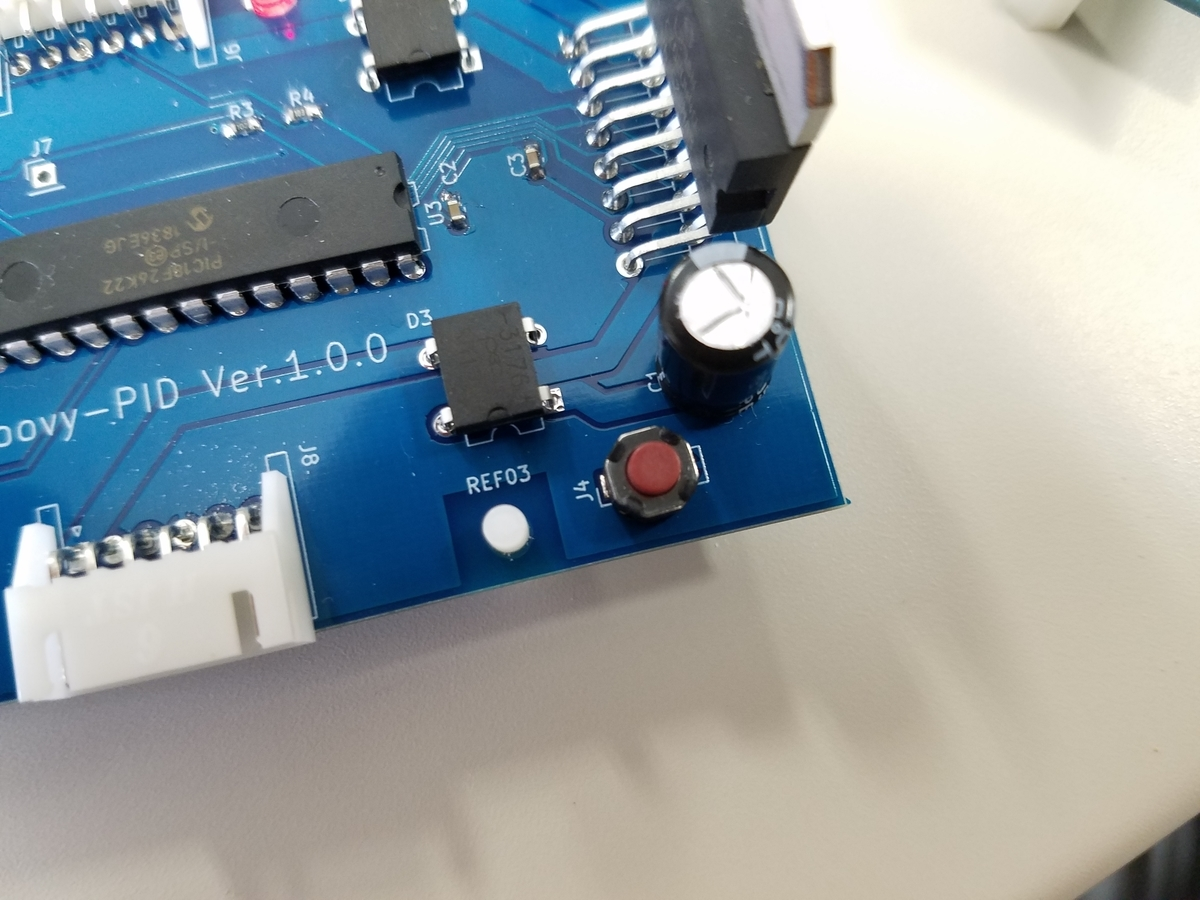Groovy-PIDのリセットスイッチを押す

```rosrun groovypid Groovy_moter_test
```

これでモータを動かすことができます

そういえばこの記事でいきなりシリアル通信してるからそれに関して少し書きたいな

# 2019/8/15(Raspberry PiのROSからpigpioを使ってGroovy-PIDを動かしてLチカしてみる)

お盆は実家でSLAMの勉強

mozyanari.hatenablog.com

このままだとロボットでは使えないので，Raspberry PiのROSからpigpioを使ってGroovy-PID上のLEDを動かしてみます

つなぎ方や動作確認，raspberry piにROSを入れることはすでに終わっている前提とします
ただ前回はGroovy IoTからPCにつなぎましたが，今回はGroovy IoTからraspberry piをUSB接続します

Raspberry Pi 3 B
OS:Ubuntu Mate
ROS:Kinetic

github.com

### 解説

```#include <pigpiod_if2.h>
pi = pigpio_start(NULL,NULL);
```

mozyanari.hatenablog.com

```char l = 0x6c;
char space = 0x20;
char zero = 0x30;
char one = 0x31;
char enter = 0x0D;
```

Groovy PIDはTeratermで送信するasciiコードで動いています
つまり，前回やったTeratermでLチカさせたコマンドは
「l」 「 」(space) 「0」「」(改行)＝ 「0x6c」「0x20」「0x30」「0x0D」
を送ればいいことになります
ここではそのコマンドを定義しています
また，シリアル通信は8bitで送受信を行うのでcharにしといたほうが安全です

```int serial_flag = serial_open(pi,"/dev/ttyACM0",57600,0);
```

ここでUSB接続したシリアルポートを開いています

##### 第一引数

pigpioを初期化した時に返ってきた値を使用しています

##### 第二引数

/dev/ttyACM0を指定していますが，他に接続している物があったり，OSのバージョンによっては違うものが出てくるかもしれませんがその時はここを適宜変更してください

##### 第三引数

Groovy-PIDを動かすのに必要なボーレートは57600なのでここはこの値で固定です

```serial_write(pi,serial_flag,&l,1);
serial_write(pi,serial_flag,&space,1);
serial_write(pi,serial_flag,&zero,1);
serial_write(pi,serial_flag,&enter,1);
```

この部分でシリアル通信でデータを送信しています

##### 第一引数

pigpioを初期化した時に返ってきた値を使用しています

##### 第二引数

シリアル通信を初期化した時の値を使用しています

### 実行

```sudo pigpiod
```

まずは絶対にこれを実行します

```roscore
```

ROSではこれが必ず必要ですGroovy-PIDのリセットスイッチを押す

```rosrun groovypid Groovy_LED_test
```

これでLEDがチカチカします

# 2019/7/29(TeratermでGroovy-PIDとGroovy IoTを動かしてみる)

ブログのアクセス数見てるとちゃんとみんな休日休んでることが分かる

www.omiya-giken.com

##### Groovy-PID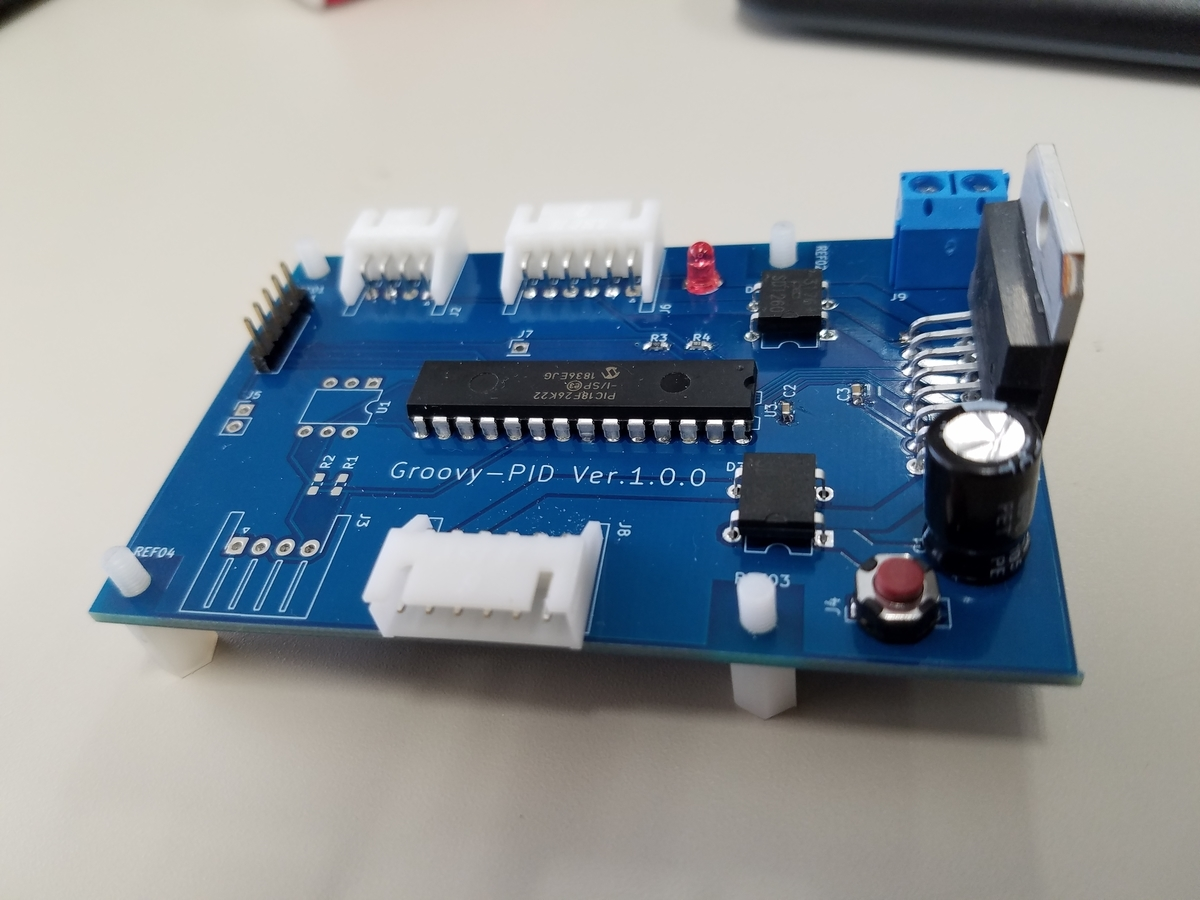www.switch-science.com

##### Groovy IoT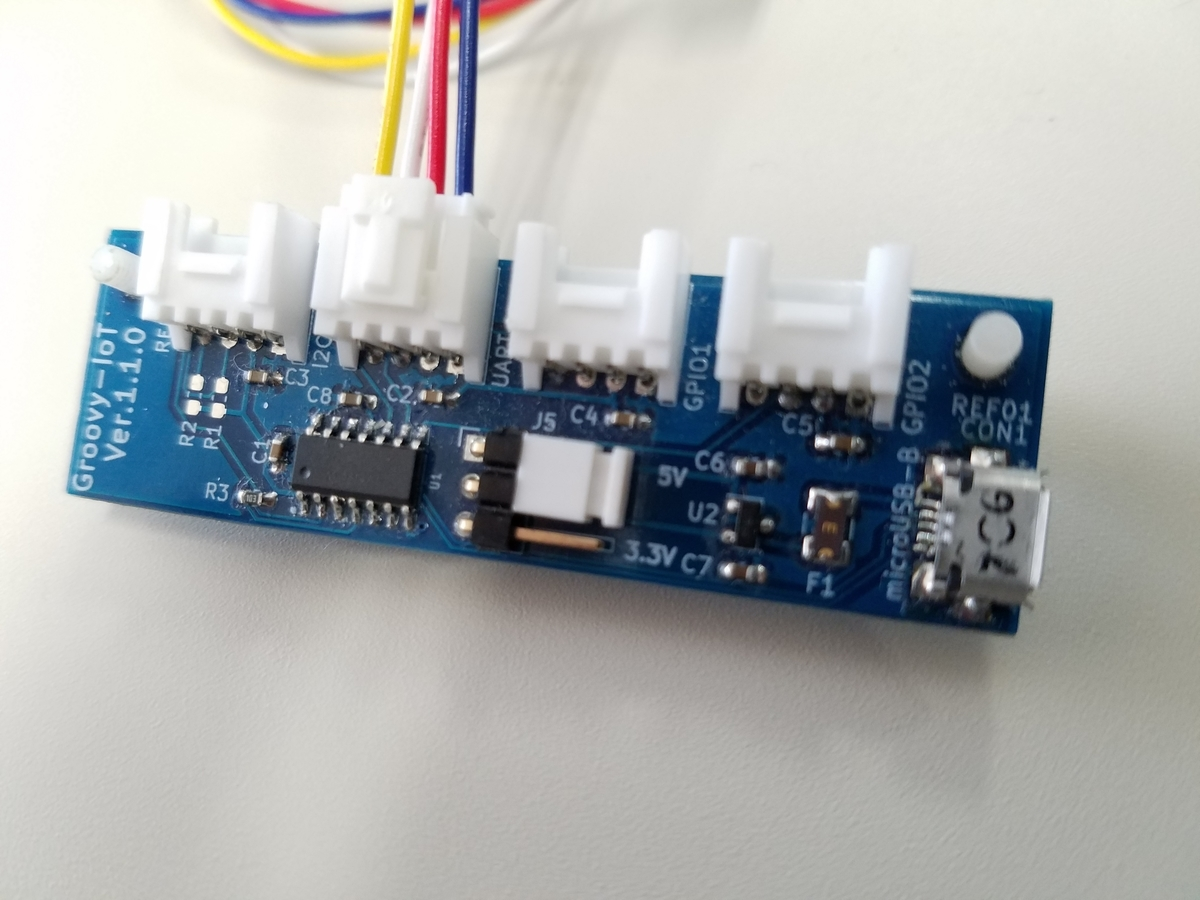www.switch-science.com

#### つなぎ方

つなぎ方は以下のページを参考にしました
www.omiya-giken.com

• Groovy-PIDとGroovy IoT間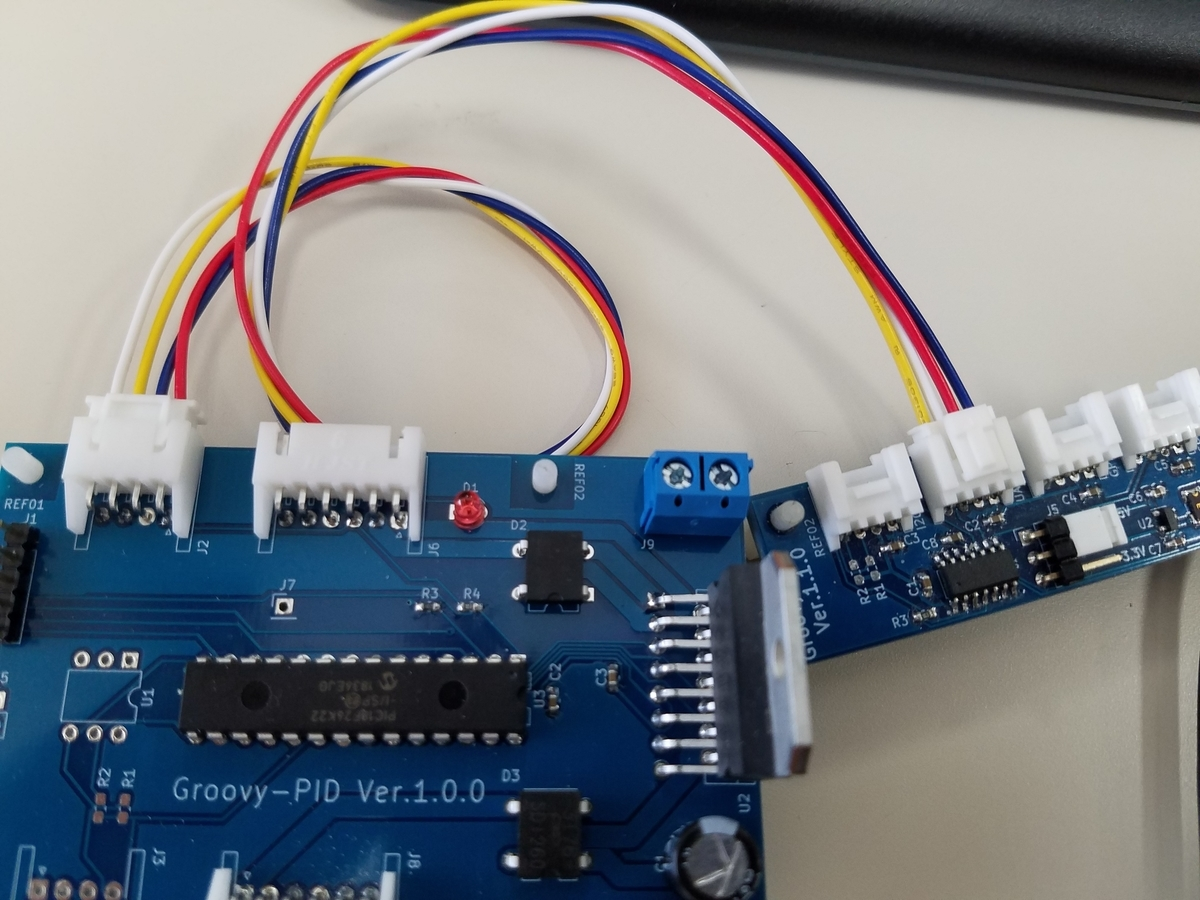4線のケーブルで接続します
ただ，ここで注意しないといけないのは，Groovy IoTには画像の左からI2C，UART，GPIO1，GPIO2の接続端子があり，UARTを使用します
(自分はここで間違ってGPIO2につないでいて小一時間動かなくて苦労しました)

• Groovy IoTとPC間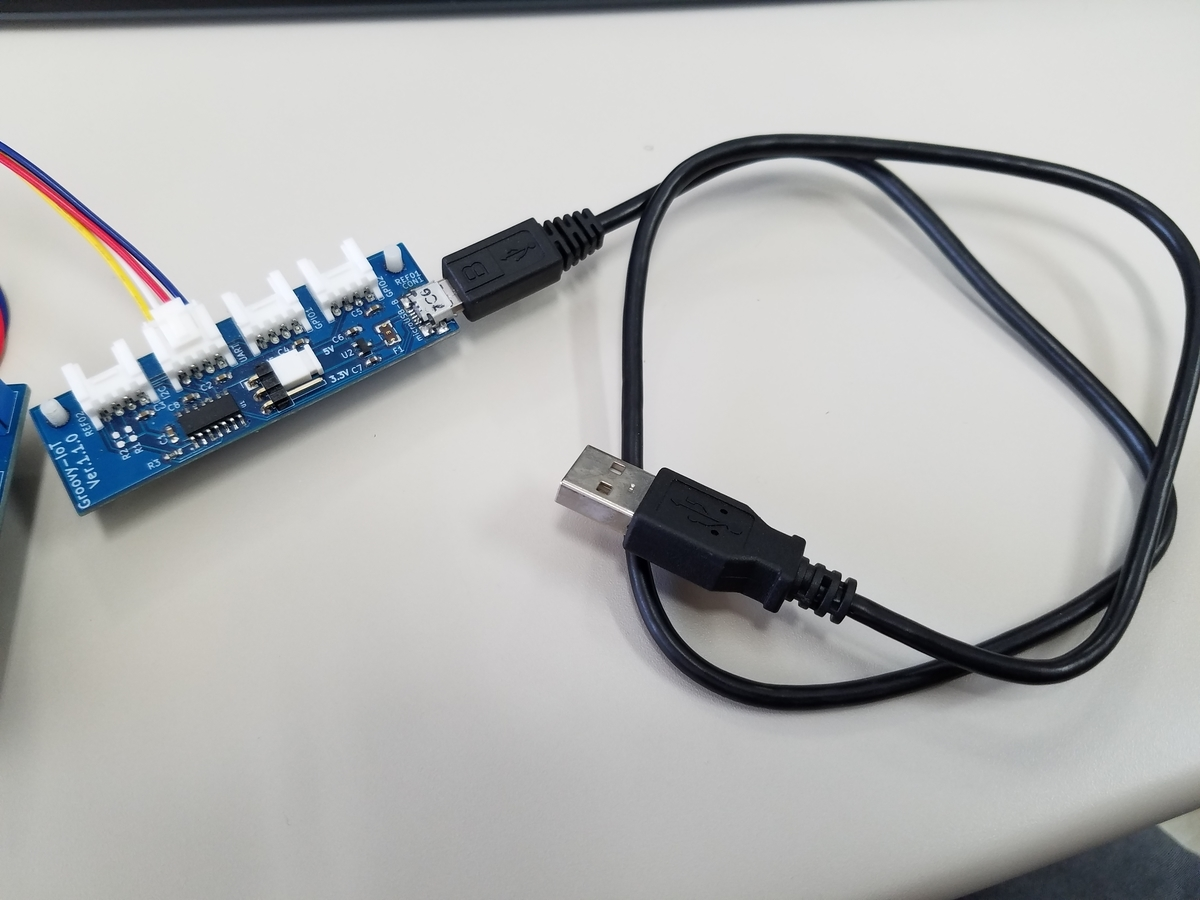microUSBでPCと接続します

#### Teratermとの接続してLEDを光らせる

www.omiya-giken.com

USB接続するとGroovy PIDの上のLEDが光ります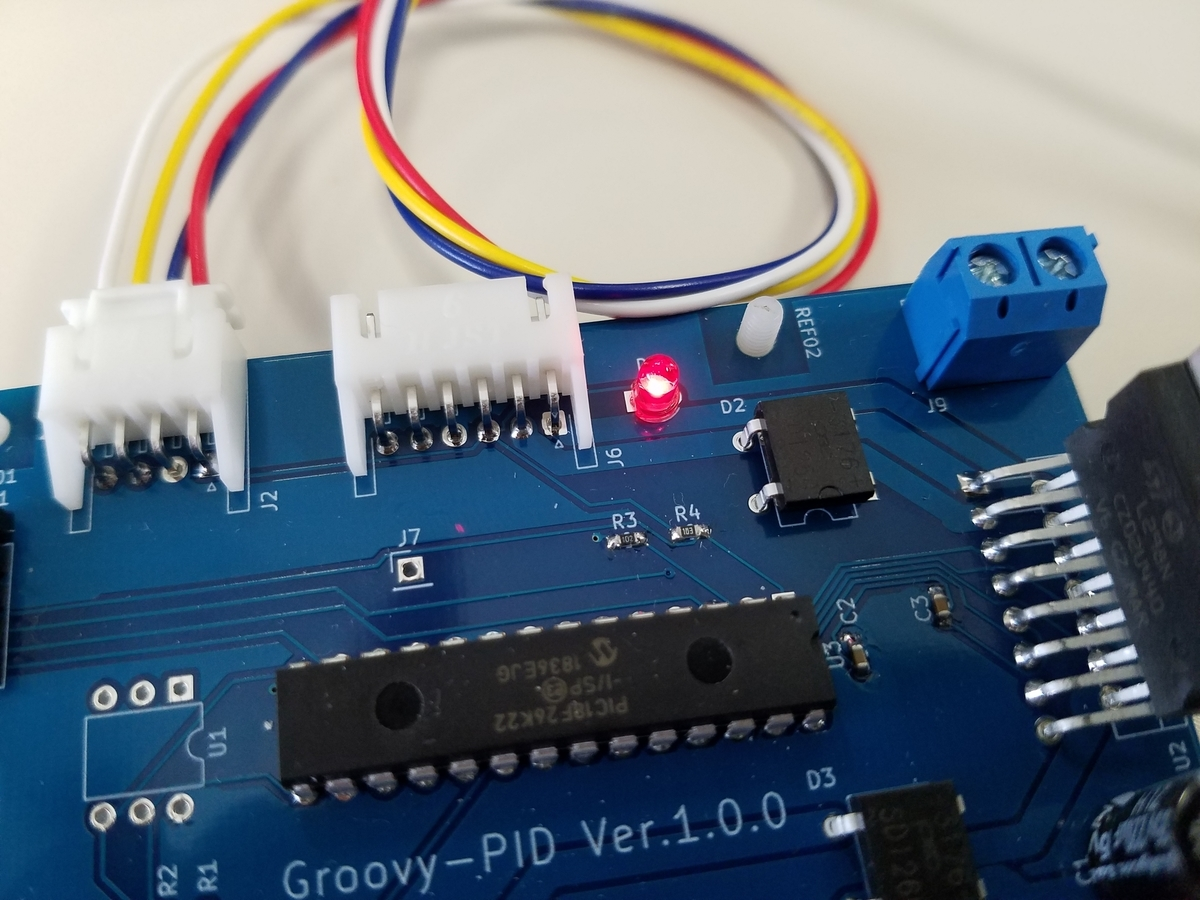Teratermを開いて接続されたCOMボードを選択します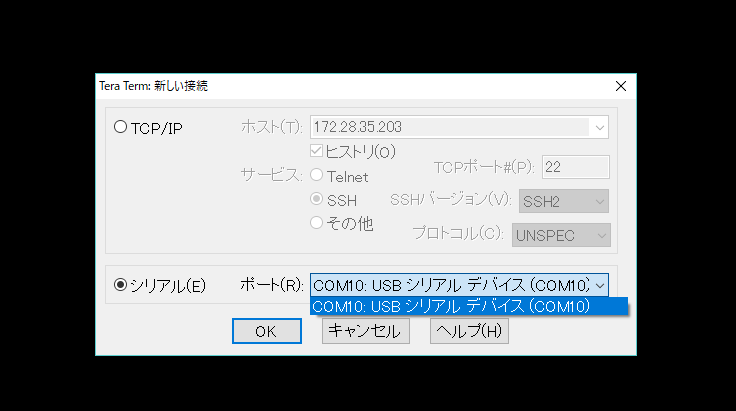ボーレートは57600，データは8bit，パリティなし，ストップbitは1にします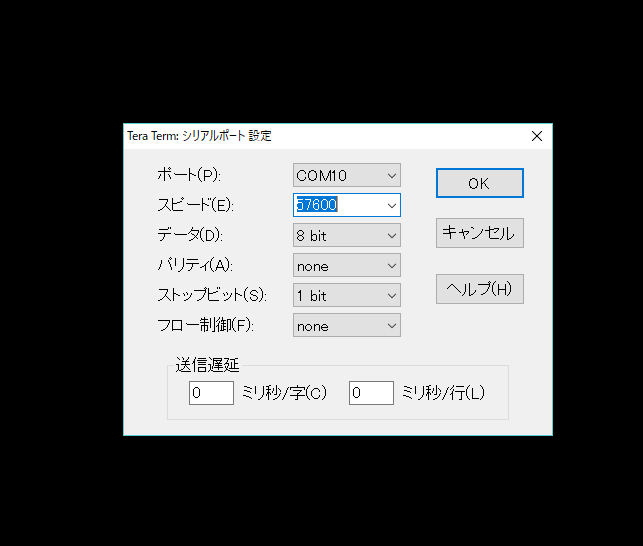このあと，Groovy PIDのリセットスイッチのJ4を押しますすると，コマンドを打てる画面が出てきます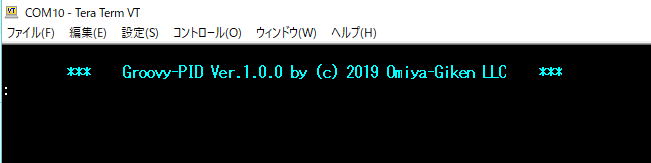「l」 「 」(space) 「0」を入力してエンターで送信します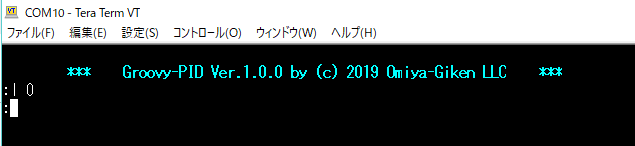すると最初は光っていたLEDが消えます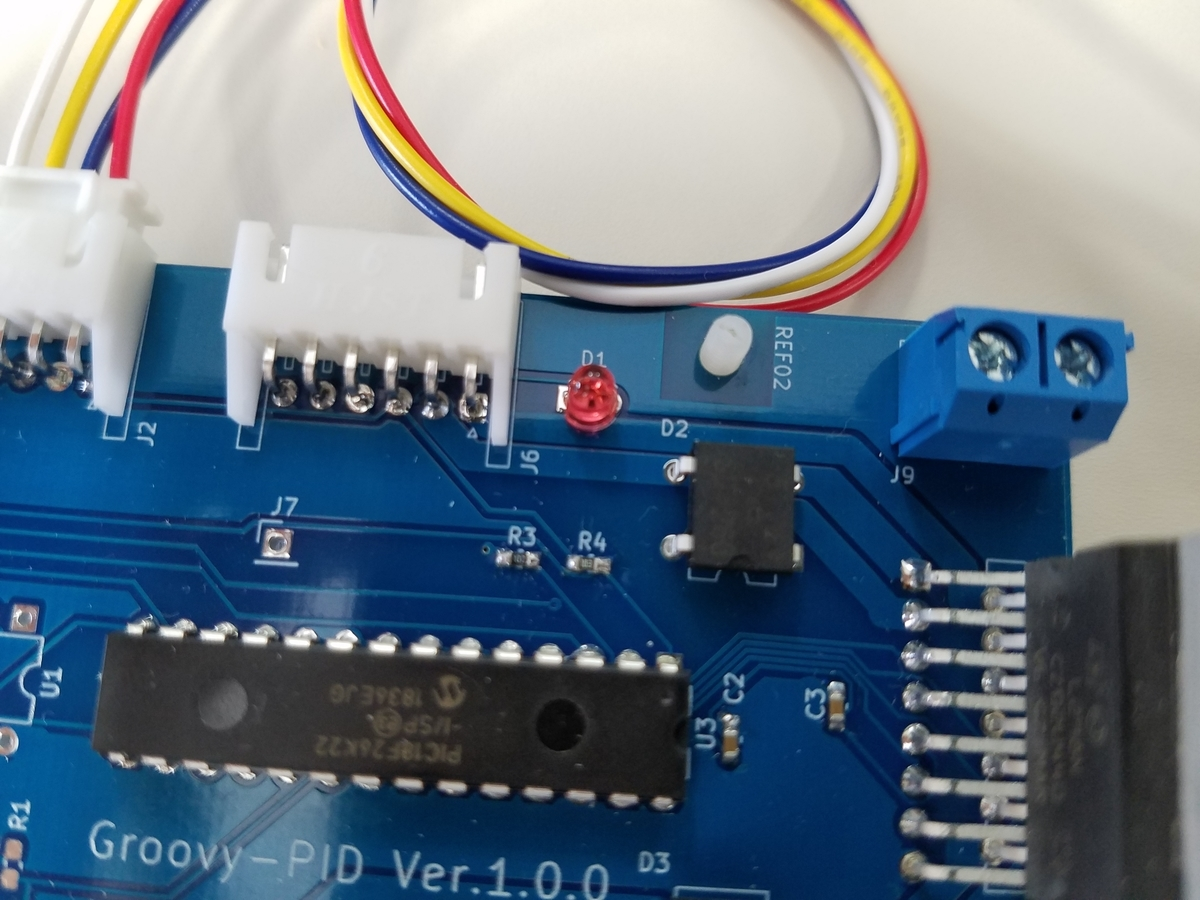これでとりあえず動くことが確認できたので終了です

# 2019/7/25(Rapberry Pi 3 + Ununtu MateでGPIOのUART(シリアル)を有効にする)

ROSでpigpioを使ってGPIOのUARTを動かそうとしたときにデフォルトの設定だとGPIOがbluetoothに使用されているらしく色々いじる必要がありました

#### 1

このページが検索結果に一番マッチしていたので，やってみました
ですがこれの最後にrebootした後，sudo pigpioをするのを忘れていてpigpioからシリアルを開こうとしても当たり前ですが開きませんでした
これで動いた人がいたら教えてほしいです

qiita.com
シリアルポートの権限付与の部分でこれだけで動いているので1のやり方だけで動く可能性があります

#### 2

www.ingenious.jp
このページによると
miniUARTがGPIO
UART0がBluetooth
につながっているらしく，Bluetoothを停止してUART0をGPIOにつなぐ，もしくはminiUARTとUART0を入れ替える必要があります

serial0→/dev/ttyS0
serial1→/dev/ttyAMA0
となっていると思います
これを入れ替えていきます

このページの手順に沿ってやってみました
Linuxのシリアルコンソールを無効化
この人との環境が少し違うので内容が少し違いました
なので，/boot/cmdline.txtを以下のように変更しました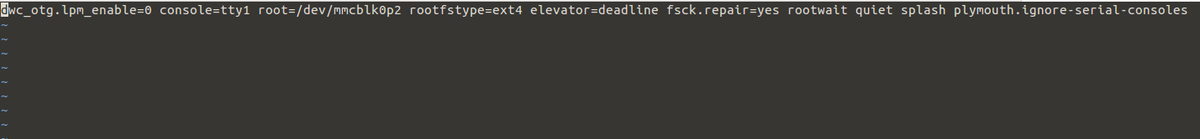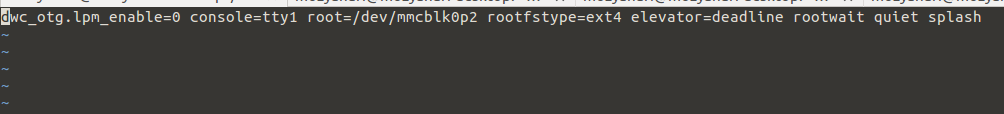②シリアル通信にUART0を使用するように変更
bluetoothは生かしたままにしたかったので
bのBluetoothとの通信にminiUARTを使用する方法を使用しました
なので，/boot/config.txtに
dtoverlay=pi3-miniuart-bt
core_freq=250
を追加しました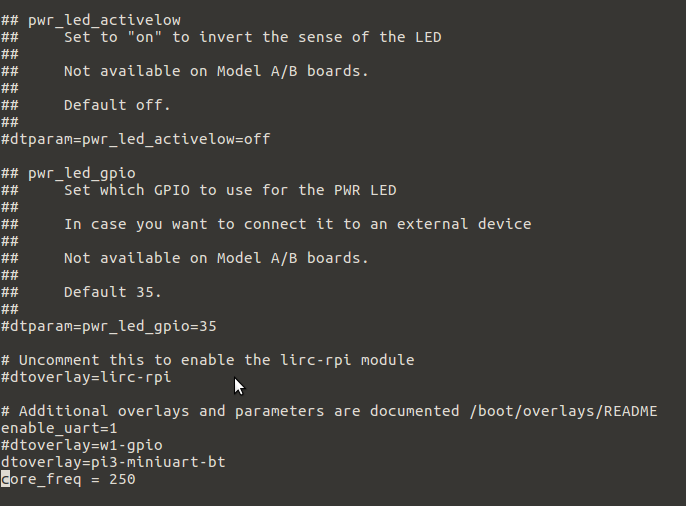こうすると，miniUARTとUART0が入れ替わります
これを確認するには
/devの中でls -lを実行すると確認ができます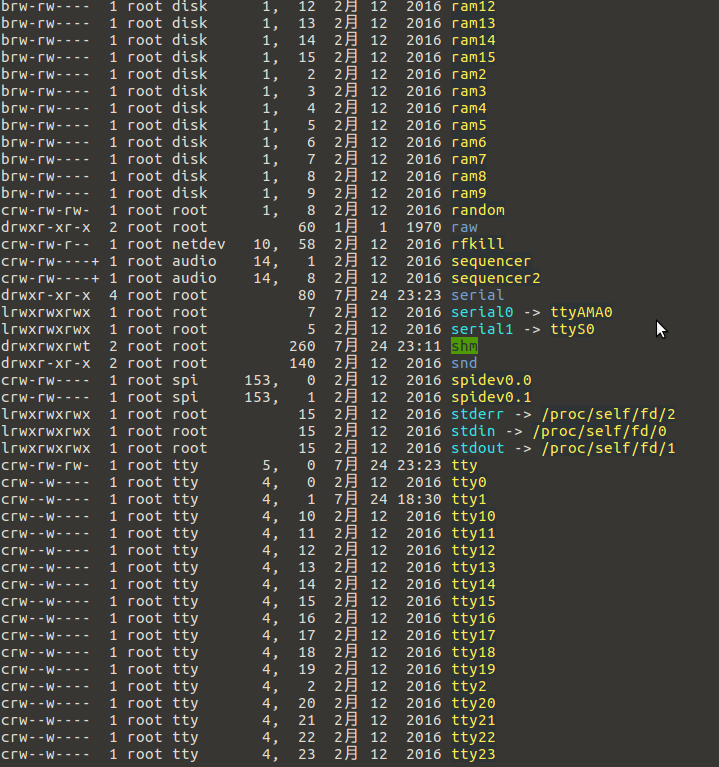miniUART =/dev/ttyS0
UART0=/dev/ttyAMA0
らしく，画像の真ん中に表示されているように
serial0→/dev/ttyAMA0
serial1→/dev/ttyS0
となればOKです

# 2019/7/25(raspberry piのROS上でpigpioを使ってGPIOからサーボを動かしてみる)

www.youtube.com

mozyanari.hatenablog.com

Lチカしてから1か月，NorthStarで遊び過ぎてこっちを忘れてました
NorthStarでやったことは，可能ならRosCon JPのLTで発表したいと思っているのでここでは書きませんが面白いものが出来たと思っています
(でもRosConのLT，企業の人がガチでやってるから学生が出来るか心配)

Lチカが出来てpigpioが正常に動いている前提で進めます

ですがサーボはたった一行で終わります

##### set_servo_pulsewidth

これを使うだけです

また，pigpioを使ったプログラムはgitにすべて挙げていますので好きなようにクローンして使ってください
github.com

#### プログラム

(前回書いた記事とは，プログラム作法が全く違いますが，前回のようにクラスまで使うまでもないと感じたのでこのように書いています)

#### 解説

```#include <pigpiod_if2.h>
pi = pigpio_start(NULL,NULL);
```

ヘッダーと初期化を行っています

```set_servo_pulsewidth(pi,26,1500);
```

abyz.me.uk

マイコンでサーボのPWMよりも簡単

##### 第二引数

ピン番号を選びます
26なのでGPIO26(ラズパイのピン番号では37)を制御します
(pigpioのいいところとしてGPIOピンのどのピンからでもPWMを出せます
これは本当に便利だし，小さいロボットならマイコンがいらない)

##### 第三引数

パルスの幅を選択します

0を入れるとPWMがストップします
リファレンスによると1500程度がサーボ中心の角度らしいです

あとはROSを理解していれば分かると思います
(分からなかったらコメントで質問ください)

#### CMakeLists.txtの編集

```add_executable(servo src/drvo.cpp)
target_link_libraries(servo \${catkin_LIBRARIES})
```

これはいつも通り必要な部分で

```target_link_libraries(servo pigpiod_if2)
```

これを絶対に忘れないように！！

#### ROSの実行

ラズパイのGPIO26にサーボをつないで，デーモンをコマンドプロンプト上で実行します

```sudo pigpiod
```

そしてrosrunで上のプログラムを実行するとサーボが1秒間隔でシャキシャキ動くと思います
arduinoよりも安定しているのか，感覚ですがサーボ特有の「ジー」っていう音が小さいように感じました

#### 検証

え？
ラズパイが本当に安定したPWM出せてるの？

だってラズパイってマイコンみたいにリアルタイム制御向いてないんじゃない？

set_servo_pulsewidth(pi,26,1500);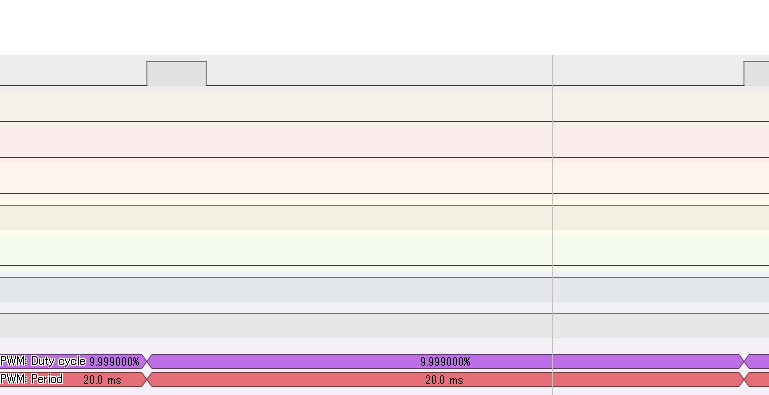set_servo_pulsewidth(pi,26,1000);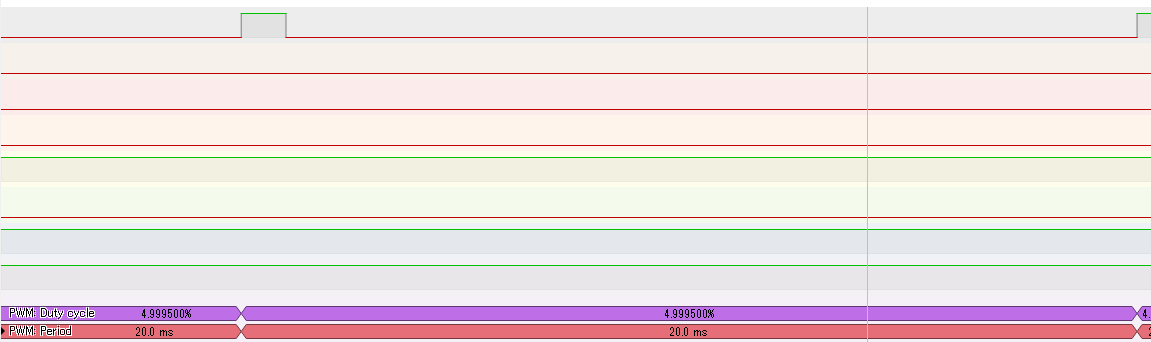#### 使っていて思うこと

pigpioとROSが連携しているので今までロボットを動かすのに必要だと考えていたマイコンを減らせるのではと考えています

ROSが入ったPC➔マイコン➔センサ・モータドライバ
だったのが
ROSが入ったラズパイ➔センサ・モータドライバ
とダイレクトに出来るのではと思います

しかもpigpioはラズパイのすべてのピンがPWM化できるのでそこらのマイコンよりは数が多いです
ただ，どれだけ同時にPWMをちゃんと出力できるか，割り込みもちゃんと使用できるか，ROSの他のノードが重い処理をするとどのような影響がピンに出てくるか，等いろいろ検討することがまだあると思います

# 2019/6/27(raspberry piのROS上でpigpioを使ってLチカしてみる)

それはROSからLEDやモータなどを動かせないということです

また，rosserial_arduinoを使えばPCからarduinoをノードとして操作できます
www.besttechnology.co.jp
www.turtlebot.com

ただ，学生ロボコンをしていた自分としては中華サーボのトルクが小さい物を動かして遊んでみたいですし，エンコーダの生値を読んでモータにPID制御をかけて発振させて遊んでみたいのです
また，arduinoしか動かせないのはスペック的につらいです

そこで目標としては，ROSが乗ったデバイスからLEDやモータを動かします
(ただROS2が出てきて組み込みマイコンにそもそもROS2が乗るのですぐオワコンになりそう)

そのためには様々な方法が現在あります

• WiringPi
• RPi.GPIO

qiita.com
ですが，自分は出来るだけPWMもあって，SPIやI2Cなどの操作もしたい
さらに，ROSから動かしたいというのがあったのでpigpioを使いました

#### pigpioのインストール

インストールは以下のページを参考にしました
karaage.hatenadiary.jp

sudo apt-get update
sudo apt-get install pigpio

#### ROS上でcppプログラムを書く

botalab.tech
またリファレンスはここです
https://abyz.me.uk/rpi/pigpio/pdif2.htmlabyz.me.ukabyz.me.uk

まずは適当にパッケージを作って，cppファイルを書きます
gpioという名前にしました

```#include <ros/ros.h>
#include <pigpiod_if2.h>

#include <std_msgs/Bool.h>

class gpio{
//construct
public:
gpio();

private:
int pi;

void cb_LED(const std_msgs::Bool::ConstPtr &data);
ros::NodeHandle nh;
ros::Subscriber sub_led;
};

gpio::gpio(){
pi = pigpio_start(NULL,NULL);

sub_led = nh.subscribe("/led", 5, &gpio::cb_LED, this);
}

void gpio::cb_LED(const std_msgs::Bool::ConstPtr &data){
if(data->data == 1){
gpio_write(pi,26,1);
}else{
gpio_write(pi,26,0);
}
}

int main(int argc, char** argv){
ros::init(argc, argv, "gpio");
gpio gpio;
ros::spin();
return 0;
}
```
##### 解説
```#include <pigpiod_if2.h>
```

ヘッダーファイルがこれです
ネットで検索しているとpythonで書かれている物ではpigpio.hをインクルードしていたりしていてるのですが何が違うかいまいち分かりません

```pi = pigpio_start(NULL,NULL);
```

ここで初期化します

ローカルで動かすのでNULLを引数として入れていますが，リファレンスを見るとIPアドレスとポートを指定できるのでもしかしたら別のPCにこのノードを立てて動かせるかも？

```gpio_write(pi,26,1);
```

piを入れている場所に負の値が入ると動きません
ここに初期化の時に帰ってきた値を使うことで初期化失敗しているときには動きません

ここでピン番号を選びます

ここではGPIOのHighかLowかを選びます
1だとHighで電圧をかけます

あとはROSを理解していれば分かると思います
(分からなかったらコメントで質問ください)

#### CMakeLists.txtの編集

```add_executable(gpio src/gpio.cpp)
target_link_libraries(gpio \${catkin_LIBRARIES})
```

を書くと思います
ただ今回は

```target_link_libraries(gpio pigpiod_if2)
```

をさらに追加する必要があります
(これが一番大事)
これを書いておかないとコンパイルが通りません

#### ROSの実行

ラズパイのGPIO26にLEDと抵抗をつないで，デーモンをコマンドプロンプト上で実行します

```sudo pigpiod
```

そして，gpioノードを実行して，/ledにtrueを送るとLEDが光ります

これでROS上でラズパイのGPIOを制御することが出来ました

# 2019/6/14(ハードウェアの干渉でNorthStarのキャリブレーションが上手くいかない)

www.youtube.com

mozyanari.hatenablog.com

NorthStarをキャリブレーションをしようとしたのですが，どれだけ値を変更しても右と左がずれていました
まあ見えているしいいかなと思っていたのですが，NorthStarを人に見せる機会があるので，このままだと体験してくれる人の感動が半減しないかと思いました

そこで，どこかハード的にいけない場所があるかなと思ってCADを見ていました
myhub.autodesk360.com

そしたら，LCDを固定する部分と本体が干渉していました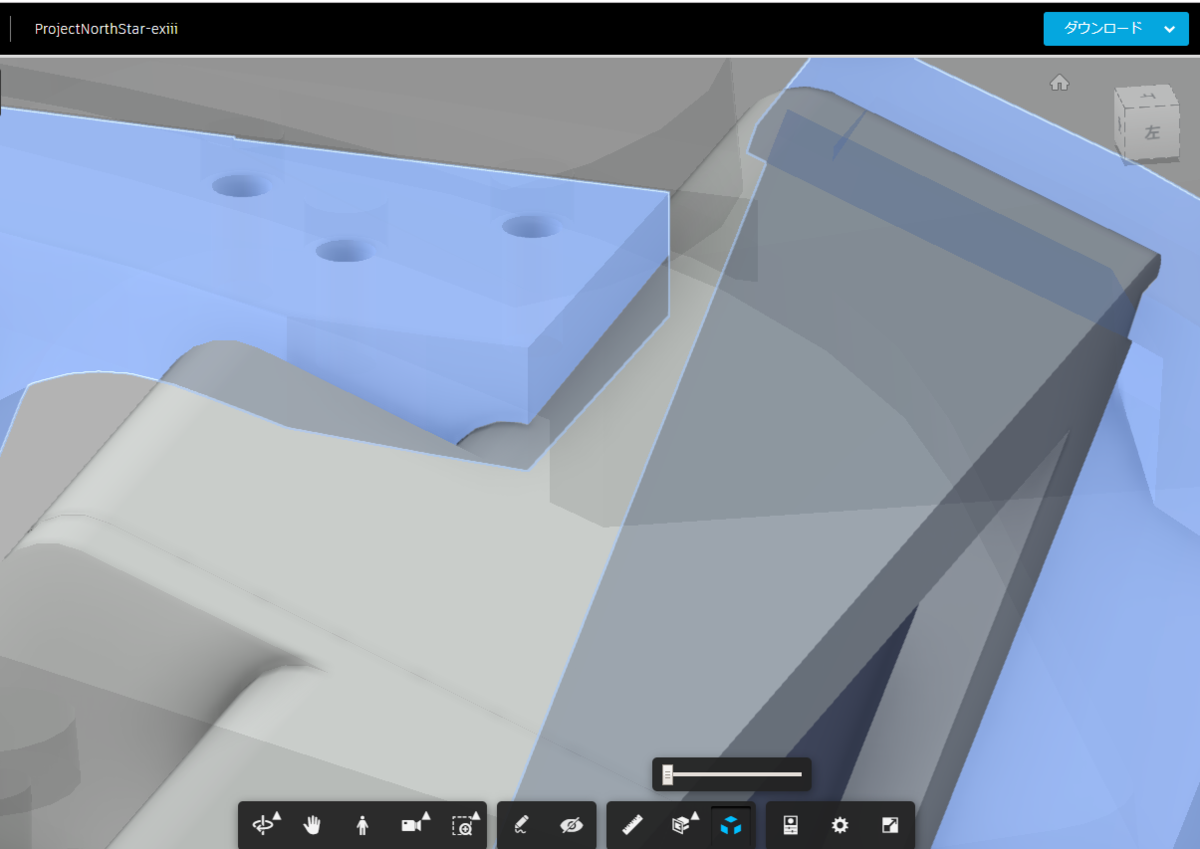おそらくこれが原因でLCDの角度が変わっていたのでしょう

# 2019/6/4(estimatePoseSingleMarkersのtvecとrvecからマーカ位置を計算する)

mozyanari.hatenablog.com
カメラ位置を計算することは出来ていましたが，自分が欲しいのはマーカの位置です
カメラ位置を計算できるということは，マーカ位置も取得できるはずなので検索しましたが全く記事が出てこなかったため色んな記事を参照しました
stackoverflow.com
www.learnopencv.com
www.learnopencv.com

• プログラム
```using System.Collections;
using System.Collections.Generic;
using UnityEngine;
using OpenCVForUnity;
using UnityEngine.SceneManagement;

public class Opencv_test : MonoBehaviour {

//カメラの番号
private int camera_number = 0;

private VideoCapture cam;
public Mat cam_frame;

private Texture2D cam_Texture;

//Arucoの設定
public ArUcoDictionary dictionaryId = ArUcoDictionary.DICT_4X4_50;
private Dictionary ar_dict;

//検出アルゴリズムの設定
private DetectorParameters detect_param;

//マーカの位置
private Mat rvecs;
private Mat tvecs;
//送信するマーカ位置
private Vector3 position;

public GameObject camera;
public GameObject marker;

// Use this for initialization
void Start () {
cam = new VideoCapture(camera_number);
//cam.Set(CaptureProperty.ConvertRgb, 3);
cam.set(16, 3);
cam_frame = new Mat();

ar_dict = Aruco.getPredefinedDictionary((int)dictionaryId);
detect_param = DetectorParameters.create();

//plopIdはopenCVの2系時に名前で定義されていたが廃止された．3系以降は番号でこれを取得する
cam_Texture = new Texture2D((int)cam.get(3), (int)cam.get(4), TextureFormat.RGB24, false);

this.GetComponent<Renderer>().material.mainTexture = cam_Texture;

tvecs = new Mat();
rvecs = new Mat();
position = new Vector3(0, 0, 0);
}

// Update is called once per frame
void Update () {
List<Mat> marker_corner = new List<Mat>(OpenCVForUnity.CvType.CV_8UC1);
Mat marker_ids = new Mat();
List<Mat> reject_points = new List<Mat>(OpenCVForUnity.CvType.CV_8UC1);

cam.read(cam_frame);

Aruco.detectMarkers(cam_frame, ar_dict, marker_corner, marker_ids, detect_param, reject_points);

//Debug.Log(marker_ids.size());
if (marker_ids.total() > 0)
{
//マーカの描画
Aruco.drawDetectedMarkers(cam_frame, marker_corner, marker_ids, new Scalar(0, 255, 0));

//キャリブレーションデータの設定
Mat camMatrix = new Mat(3, 3, CvType.CV_64FC1);
camMatrix.put(0, 0, 548.82998077504431);
camMatrix.put(0, 1, 0);
camMatrix.put(0, 2, 332.15854329853039);
camMatrix.put(1, 0, 0);
camMatrix.put(1, 1, 549.82809802204554);
camMatrix.put(1, 2, 236.24689239842121);
camMatrix.put(2, 0, 0);
camMatrix.put(2, 1, 0);
camMatrix.put(2, 2, 1.0f);

MatOfDouble distCoeffs = new MatOfDouble(-0.088668381409835462, 0.4802348866900254, -0.0026481695882058297, 0.0019686217165377452, -0.82389338772252729);

Aruco.estimatePoseSingleMarkers(marker_corner, (float)0.125, camMatrix, distCoeffs, rvecs, tvecs);

Aruco.drawAxis(cam_frame, camMatrix, distCoeffs, rvecs, tvecs, (float)0.1);

Debug.Log("x=" + tvecs.get(0, 0) + "y=" + tvecs.get(0, 0) + "z=" + tvecs.get(0, 0));
//Debug.Log("y=" + tvecs.get(0, 0));
//Debug.Log("z=" + tvecs.get(0, 0));

Debug.Log("roll=" + (rvecs.get(0, 0) * 180 / Mathf.PI) + "pitch=" + rvecs.get(0, 0) * 180 / Mathf.PI + "yaw=" + rvecs.get(0, 0) * 180 / Mathf.PI);

marker.transform.position = new Vector3(0, 0, 0);

Mat Rt = new Mat();

OpenCVForUnity.Calib3d.Rodrigues(rvecs, Rt);

Mat T = new Mat(3, 1, CvType.CV_64FC1);
T.put(0, 0, tvecs.get(0, 0));
T.put(1, 0, tvecs.get(0, 0));
T.put(2, 0, tvecs.get(0, 0));

//Debug.Log(R);

Mat R = Rt.t();

Mat camera_position = Rt.t() * (-T);

Debug.Log(camera_position);

//回転角度の計算
float roll = 0;
float pitch = 0;
float yaw = 0;

float a = Mathf.Sqrt((float)(R.get(0, 0) * R.get(0, 0) + R.get(1, 0) * R.get(1, 0)));
if (a > 1e-6)
{
roll = Mathf.Atan2((float)R.get(2, 1), (float)R.get(2, 2)) * 180 / Mathf.PI;
pitch = Mathf.Atan2((-(float)R.get(2, 0)) , a) * 180 / Mathf.PI;
yaw = Mathf.Atan2((float)R.get(1, 0), (float)R.get(0, 0)) * 180 / Mathf.PI;
}
else
{
roll = Mathf.Atan2(-(float)R.get(1, 2), (float)R.get(1, 1)) * 180 / Mathf.PI;
pitch = Mathf.Atan2((-(float)R.get(2, 0)) , a) * 180 / Mathf.PI;
yaw = 0;
}

camera.transform.position = new Vector3((float)tvecs.get(0, 0) * 10, -(float)tvecs.get(0, 0) * 10, (float)tvecs.get(0, 0) * 10);

camera.transform.rotation = Quaternion.Euler(roll, pitch, yaw);

}

Utils.matToTexture2D(cam_frame, cam_Texture);
}

public enum ArUcoDictionary
{
DICT_4X4_50 = Aruco.DICT_4X4_50,
DICT_4X4_100 = Aruco.DICT_4X4_100,
DICT_4X4_250 = Aruco.DICT_4X4_250,
DICT_4X4_1000 = Aruco.DICT_4X4_1000,
DICT_5X5_50 = Aruco.DICT_5X5_50,
DICT_5X5_100 = Aruco.DICT_5X5_100,
DICT_5X5_250 = Aruco.DICT_5X5_250,
DICT_5X5_1000 = Aruco.DICT_5X5_1000,
DICT_6X6_50 = Aruco.DICT_6X6_50,
DICT_6X6_100 = Aruco.DICT_6X6_100,
DICT_6X6_250 = Aruco.DICT_6X6_250,
DICT_6X6_1000 = Aruco.DICT_6X6_1000,
DICT_7X7_50 = Aruco.DICT_7X7_50,
DICT_7X7_100 = Aruco.DICT_7X7_100,
DICT_7X7_250 = Aruco.DICT_7X7_250,
DICT_7X7_1000 = Aruco.DICT_7X7_1000,
DICT_ARUCO_ORIGINAL = Aruco.DICT_ARUCO_ORIGINAL,
}

void OnDestroy()
{
if (cam_frame != null)
cam_frame.Dispose();
}

}
```

• マーカ位置の計算

tvecとrvecを取得するまでは前回の記事と同じ流れです

```camera.transform.position = new Vector3((float)tvecs.get(0, 0) * 10, -(float)tvecs.get(0, 0) * 10, (float)tvecs.get(0, 0) * 10);
```

10倍してるのはデバックするときに動きが見やすいためにしています

• マーカの角度計算
```float roll = 0;
float pitch = 0;
float yaw = 0;

float a = Mathf.Sqrt((float)(R.get(0, 0) * R.get(0, 0) + R.get(1, 0) * R.get(1, 0)));
if (a > 1e-6)
{
roll = Mathf.Atan2((float)R.get(2, 1), (float)R.get(2, 2)) * 180 / Mathf.PI;
pitch = Mathf.Atan2((-(float)R.get(2, 0)) , a) * 180 / Mathf.PI;
yaw = Mathf.Atan2((float)R.get(1, 0), (float)R.get(0, 0)) * 180 / Mathf.PI;
}else{
roll = Mathf.Atan2(-(float)R.get(1, 2), (float)R.get(1, 1)) * 180 / Mathf.PI;
pitch = Mathf.Atan2((-(float)R.get(2, 0)) , a) * 180 / Mathf.PI;
yaw = 0;
}
```

この部分が最も時間がかかりました
rvecsをロドリゲス変換して転置した後の行列の中身を参考にしたページをもとに計算するとなんかうまいこと動くようになりました
なので，なぜこれが上手く動くか理解していません

# 2019/6/4(estimatePoseSingleMarkersのtvecとrvecからカメラ姿勢を計算する)

ココロオークションが最近の自分の流行
www.youtube.com

Arucoを使ってマーカの位置を取得しようとしています
Unityでマーカ位置にオブジェクトを置きたいのでちゃんとした位置結果を計算しないといけません
ネットで検索しても，detectMarkers→drawDetectedMarkersで描画するだけや，detectMarkers→tvecの値から直接位置計算をやっていて正解にたどり着けませんでした

OpencvにestimatePoseSingleMarkersと同様の結果を出すsolvePnPという関数があり，これで検索すれば出てくるかなと思って検索して見つけました
stackoverflow.com
これによって自分が出来たのはマーカを原点としてカメラの位置・姿勢がどこにあるかということです．

• 環境

Unity 2018.2.21f1
OpenCV for Unity v2.3.2

• プログラム
```using System.Collections;
using System.Collections.Generic;
using UnityEngine;
using OpenCVForUnity;
using UnityEngine.SceneManagement;

public class Opencv_test : MonoBehaviour {

//カメラの番号
private int camera_number = 0;

private VideoCapture cam;
public Mat cam_frame;

private Texture2D cam_Texture;

//Arucoの設定
public ArUcoDictionary dictionaryId = ArUcoDictionary.DICT_4X4_50;
private Dictionary ar_dict;

//検出アルゴリズムの設定
private DetectorParameters detect_param;

//マーカの位置
private Mat rvecs;
private Mat tvecs;
//送信するマーカ位置
private Vector3 position;

public GameObject camera;
public GameObject marker;

// Use this for initialization
void Start () {
cam = new VideoCapture(camera_number);
//cam.Set(CaptureProperty.ConvertRgb, 3);
cam.set(16, 3);
cam_frame = new Mat();

ar_dict = Aruco.getPredefinedDictionary((int)dictionaryId);
detect_param = DetectorParameters.create();

//plopIdはopenCVの2系時に名前で定義されていたが廃止された．3系以降は番号でこれを取得する
cam_Texture = new Texture2D((int)cam.get(3), (int)cam.get(4), TextureFormat.RGB24, false);

this.GetComponent<Renderer>().material.mainTexture = cam_Texture;

tvecs = new Mat();
rvecs = new Mat();
position = new Vector3(0, 0, 0);
}

// Update is called once per frame
void Update () {
List<Mat> marker_corner = new List<Mat>(OpenCVForUnity.CvType.CV_8UC1);
Mat marker_ids = new Mat();
List<Mat> reject_points = new List<Mat>(OpenCVForUnity.CvType.CV_8UC1);

cam.read(cam_frame);

Aruco.detectMarkers(cam_frame, ar_dict, marker_corner, marker_ids, detect_param, reject_points);

//Debug.Log(marker_ids.size());
if (marker_ids.total() > 0)
{
//マーカの描画
Aruco.drawDetectedMarkers(cam_frame, marker_corner, marker_ids, new Scalar(0, 255, 0));

//キャリブレーションデータの設定
Mat camMatrix = new Mat(3, 3, CvType.CV_64FC1);
camMatrix.put(0, 0, 548.82998077504431);
camMatrix.put(0, 1, 0);
camMatrix.put(0, 2, 332.15854329853039);
camMatrix.put(1, 0, 0);
camMatrix.put(1, 1, 549.82809802204554);
camMatrix.put(1, 2, 236.24689239842121);
camMatrix.put(2, 0, 0);
camMatrix.put(2, 1, 0);
camMatrix.put(2, 2, 1.0f);

MatOfDouble distCoeffs = new MatOfDouble(-0.088668381409835462, 0.4802348866900254, -0.0026481695882058297, 0.0019686217165377452, -0.82389338772252729);

Aruco.estimatePoseSingleMarkers(marker_corner, (float)0.125, camMatrix, distCoeffs, rvecs, tvecs);

Debug.Log("x=" + tvecs.get(0, 0)+ "y=" + tvecs.get(0, 0) + "z=" + tvecs.get(0, 0));

Debug.Log("roll=" + (rvecs.get(0, 0) * 180 / Mathf.PI) + "pitch=" + rvecs.get(0, 0) * 180 / Mathf.PI + "yaw=" + rvecs.get(0, 0) * 180 / Mathf.PI);

marker.transform.position = new Vector3(0, 0, 0);
Mat Rt = new Mat();

OpenCVForUnity.Calib3d.Rodrigues(rvecs,Rt);

Mat T = new Mat(3, 1, CvType.CV_64FC1);
T.put(0, 0, tvecs.get(0, 0));
T.put(1, 0, tvecs.get(0, 0));
T.put(2, 0, tvecs.get(0, 0));

Mat R = Rt.t();

Mat camera_position = Rt.t() * (-T);

Debug.Log(camera_position);

camera.transform.position = new Vector3((float)camera_position.get(0,0)*10, (float)camera_position.get(2, 0)*10, (float)camera_position.get(1, 0)*10);

float roll = Mathf.Atan2((float)-R.get(2, 1), (float)R.get(2, 2)) * 180 / Mathf.PI;
float pitch = Mathf.Asin((float)R.get(2, 0)) * 180 / Mathf.PI;
float yaw = Mathf.Atan2((float)-R.get(1, 0), (float)R.get(0, 0)) * 180 / Mathf.PI;

camera.transform.rotation = Quaternion.Euler(roll, yaw, pitch);

}

Utils.matToTexture2D(cam_frame, cam_Texture);
}

public enum ArUcoDictionary
{
DICT_4X4_50 = Aruco.DICT_4X4_50,
DICT_4X4_100 = Aruco.DICT_4X4_100,
DICT_4X4_250 = Aruco.DICT_4X4_250,
DICT_4X4_1000 = Aruco.DICT_4X4_1000,
DICT_5X5_50 = Aruco.DICT_5X5_50,
DICT_5X5_100 = Aruco.DICT_5X5_100,
DICT_5X5_250 = Aruco.DICT_5X5_250,
DICT_5X5_1000 = Aruco.DICT_5X5_1000,
DICT_6X6_50 = Aruco.DICT_6X6_50,
DICT_6X6_100 = Aruco.DICT_6X6_100,
DICT_6X6_250 = Aruco.DICT_6X6_250,
DICT_6X6_1000 = Aruco.DICT_6X6_1000,
DICT_7X7_50 = Aruco.DICT_7X7_50,
DICT_7X7_100 = Aruco.DICT_7X7_100,
DICT_7X7_250 = Aruco.DICT_7X7_250,
DICT_7X7_1000 = Aruco.DICT_7X7_1000,
DICT_ARUCO_ORIGINAL = Aruco.DICT_ARUCO_ORIGINAL,
}

void OnDestroy()
{
if (cam_frame != null)
cam_frame.Dispose();
}

}
```
• ARマーカの認識
```Aruco.detectMarkers(cam_frame, ar_dict, marker_corner, marker_ids, detect_param, reject_points);
```

cam_frameがカメラ画像でこれを入力することで，マーカの位置のmarker_cornerを取得します

• ARマーカの位置の取得
```Aruco.estimatePoseSingleMarkers(marker_corner, (float)0.125, camMatrix, distCoeffs, rvecs, tvecs);
```

マーカ位置のmarker_cornerとカメラパラメータのcamMatrixとdistCoeffsを入力します
カメラパラメータは事前に取得しておきます
mozyanari.hatenablog.com
(float)0.125はARマーカの実際の大きさをはかって入力しています
こうすることで，ARマーカとの相対的な位置関係を表す，tvecとrvecを取得出来ます

• rvecとtvecからカメラ位置を計算

ロドリゲス変換

```OpenCVForUnity.Calib3d.Rodrigues(rvecs,Rt);
```

カメラ位置の計算

```Mat camera_position = Rt.t() * (-T);
```

カメラの回転角度計算

```float roll = Mathf.Atan2((float)-R.get(2, 1), (float)R.get(2, 2)) * 180 / Mathf.PI;
float pitch = Mathf.Asin((float)R.get(2, 0)) * 180 / Mathf.PI;
float yaw = Mathf.Atan2((float)-R.get(1, 0), (float)R.get(0, 0)) * 180 / Mathf.PI;
```

Unityに適した形で入力

```camera.transform.rotation = Quaternion.Euler(roll, yaw, pitch);
```

これによってマーカの位置を原点として，カメラの位置・姿勢を取得できると思います

マーカ位置を計算するVerも書きました
mozyanari.hatenablog.com

# 2019/5/3(ArucoUnityでCharuco boardを作ってカメラのキャリブレーションデータを取得する)

mozyanari.hatenablog.com

Assets/Scenesの中のCreateMakers.unityを開きます

なので，そのままUnityの実行ボタンを押します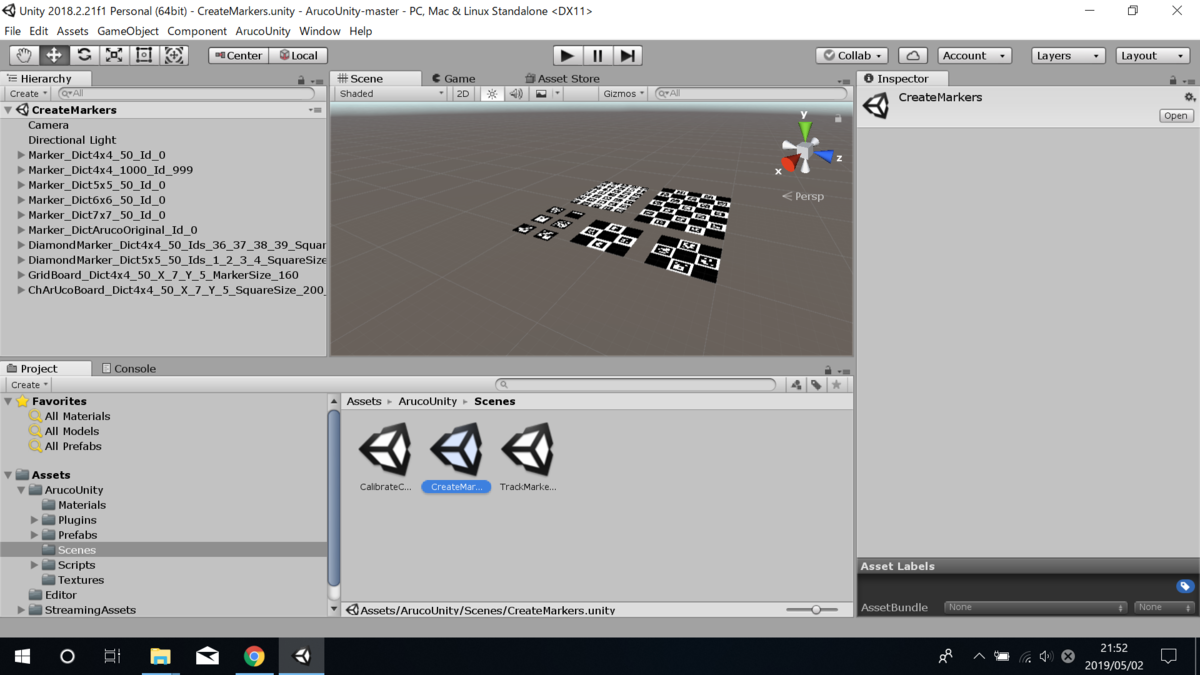すると，Assets/Imagesフォルダが生成されて，キャリブレーション用のCharuco boardが作れます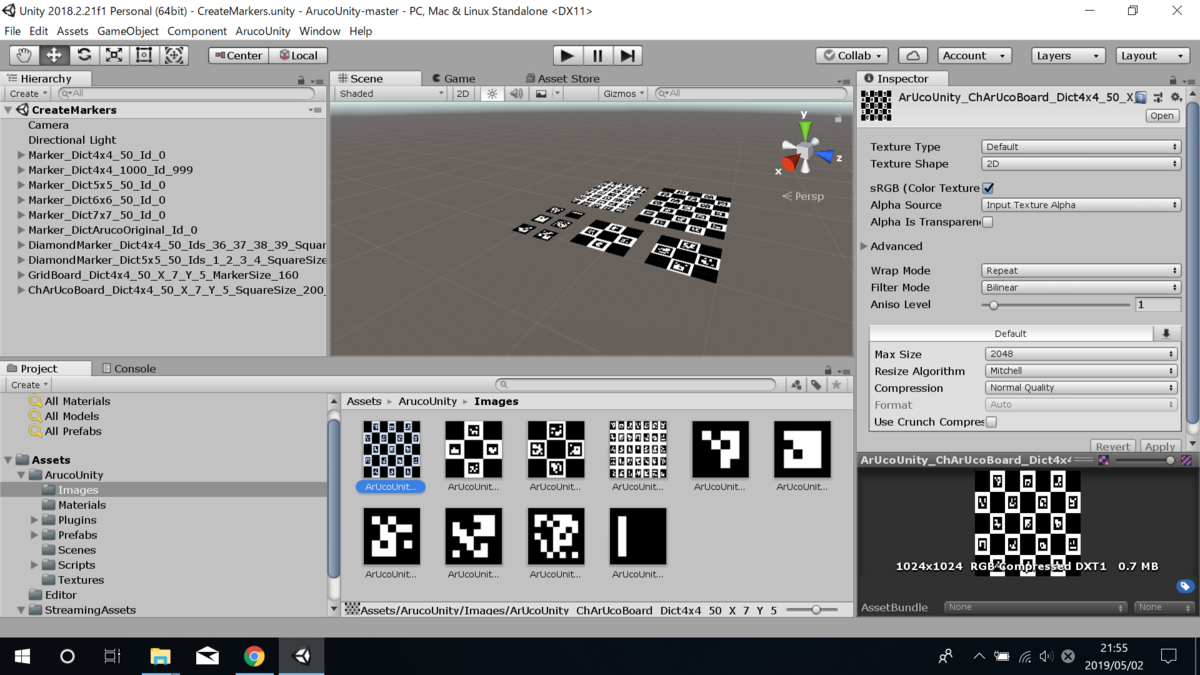ArUcoUnity_ChArUcoBoard_Dict4x4_50_X_7_Y_5_SquareSize_200_MarkerSize_120.png
です

これを印刷して厚紙に貼り付けます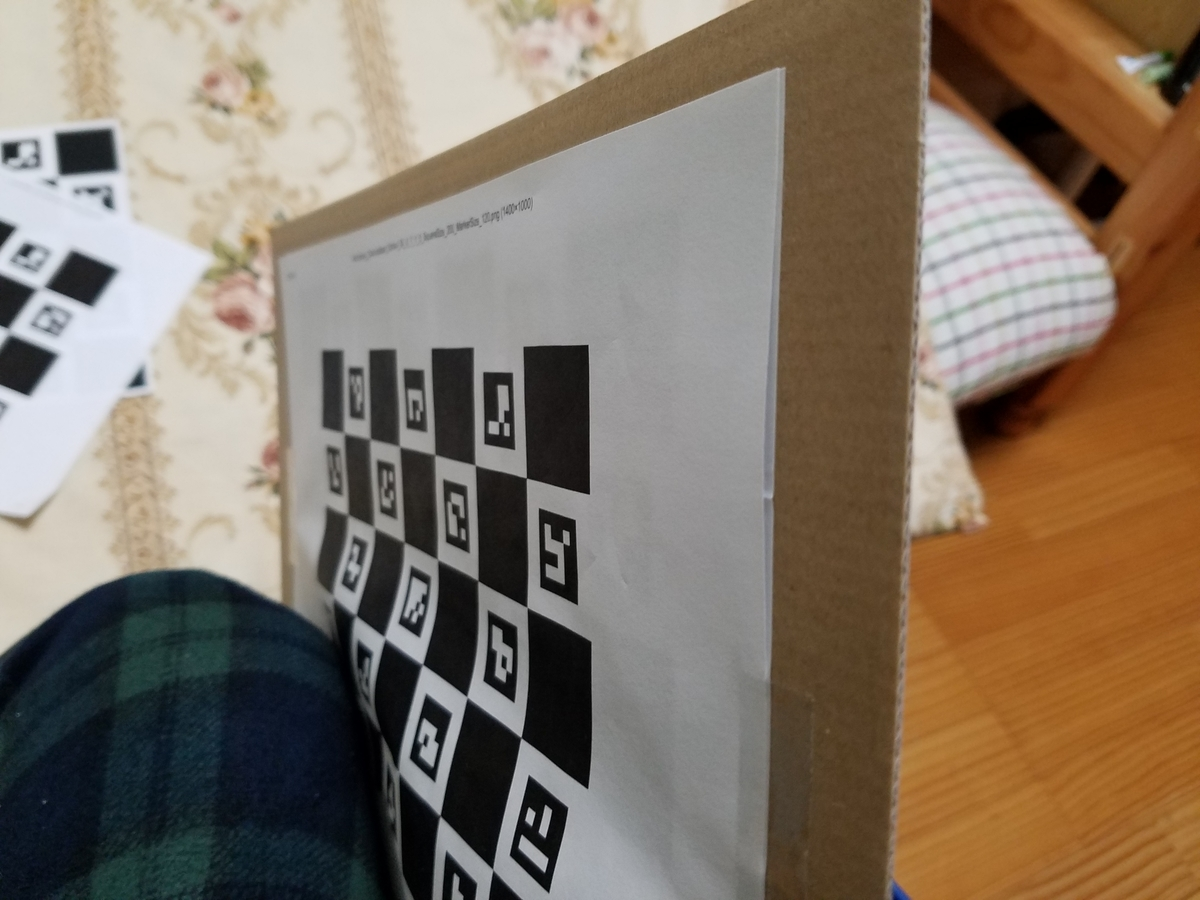Assets/Scenesの中のCalibrateCamera.unityを開きます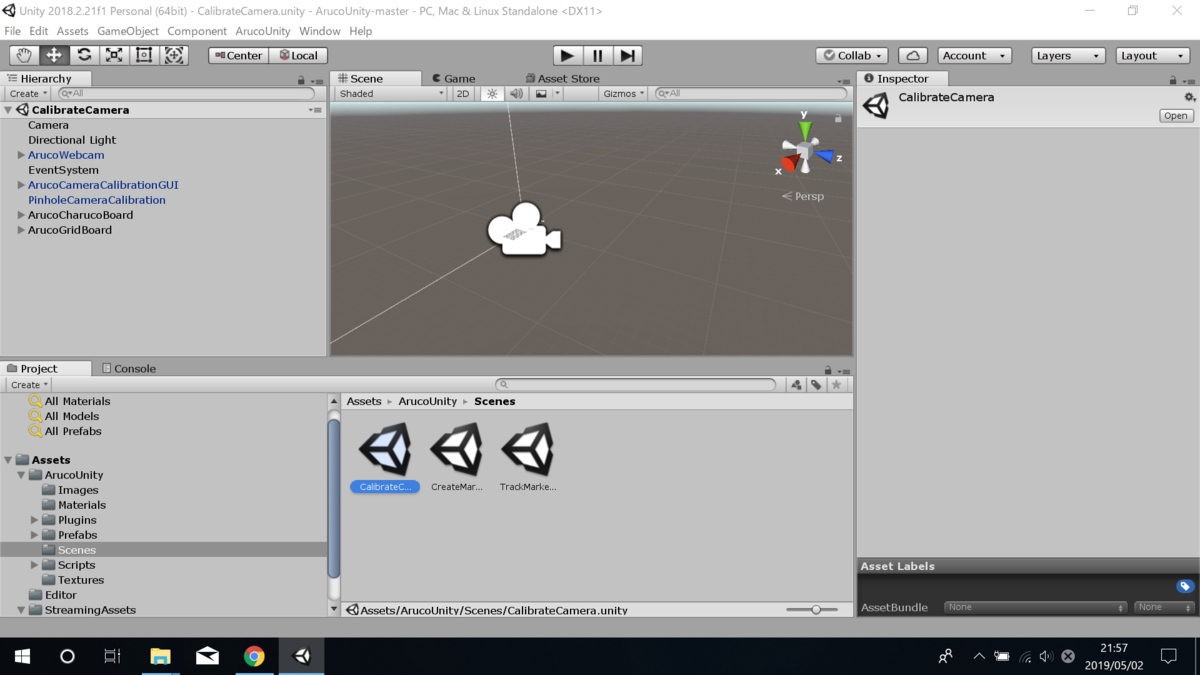まず指定するのは使うカメラです
Aruco WebcamWebcam Idを設定します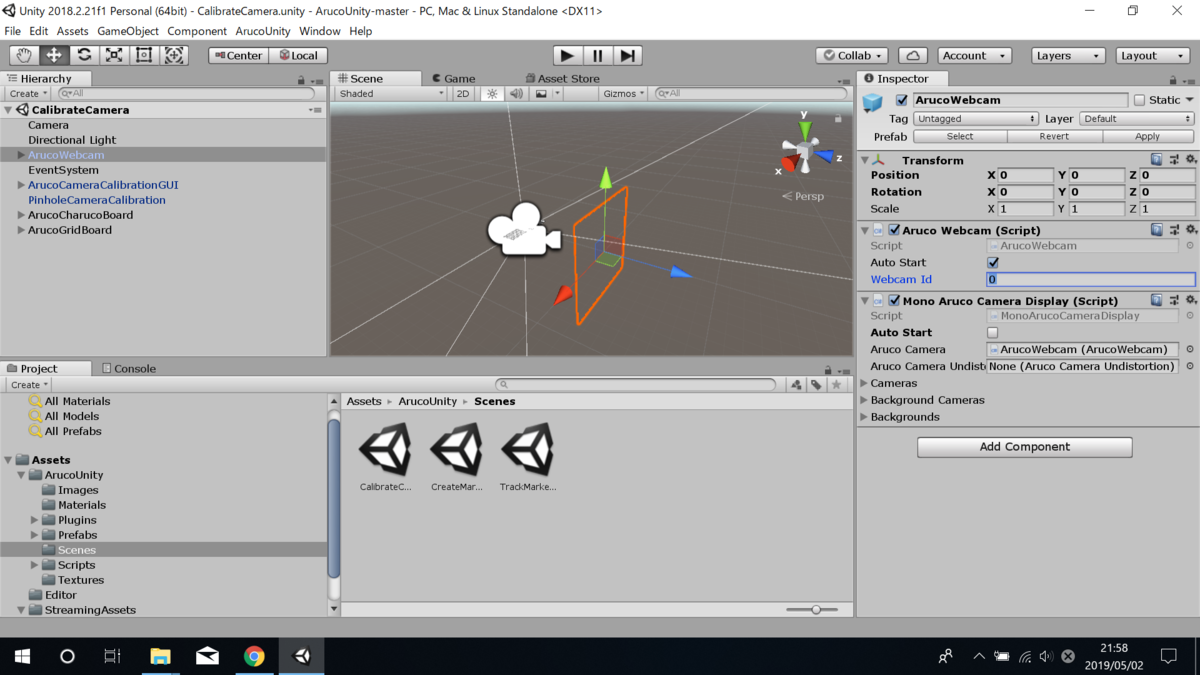Mono Aruco Camera Displayのチェックボックスを消します
キャリブレーション実行時にこれが起動しないようにするためらしいです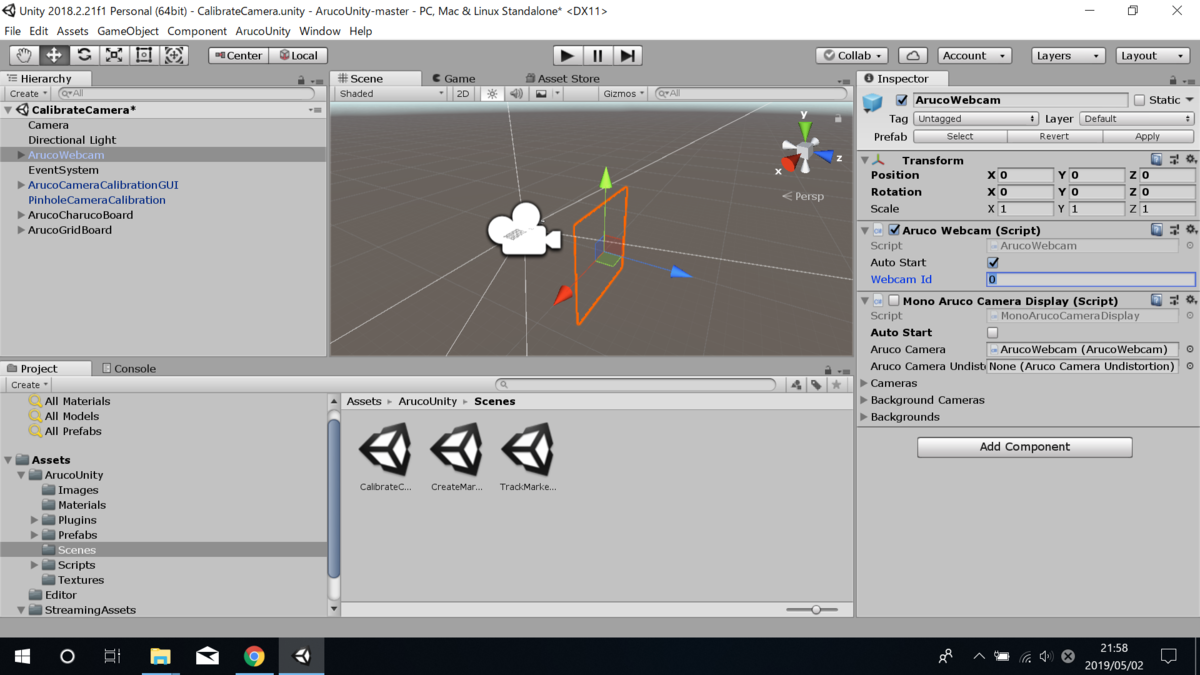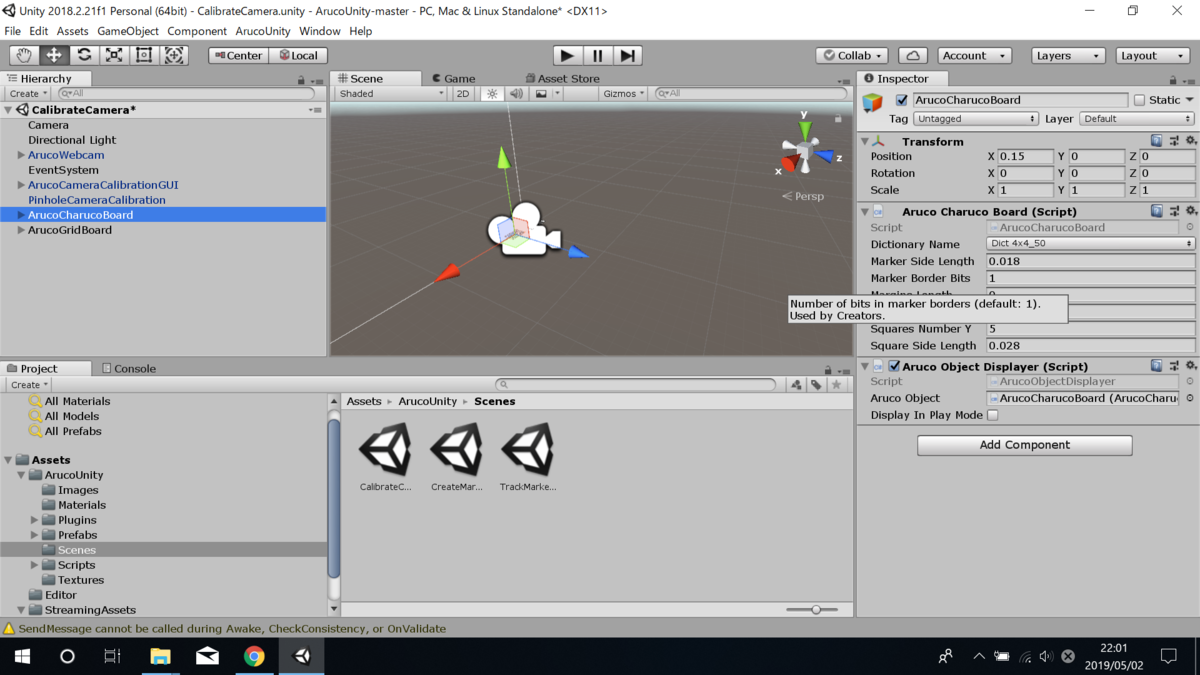Maker Side Lengthはマーカの大きさを，Square Side LengthはQRコードの一つのマスの大きさをm単位で指定します
なので，自分が印刷したCharuco boardを定規で測って入力してください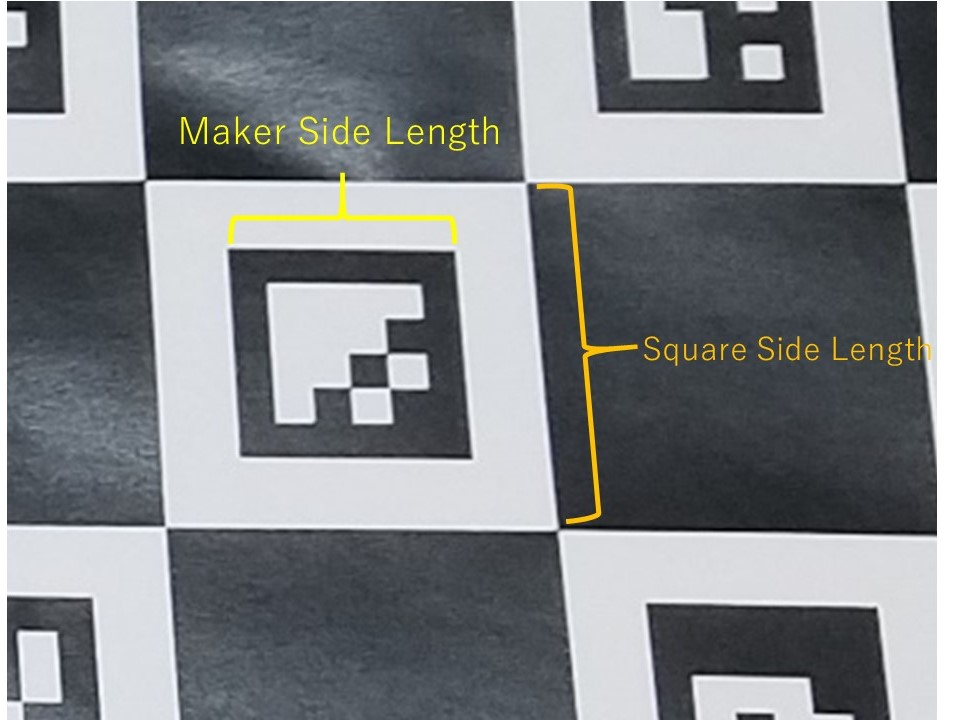(こうすることで，印刷機が勝手にCharuco boardを拡大したり縮小したりして印刷しても大丈夫になっています)

Camera Parameterに，「自分が好きな名前.xml」を入力してください
キャリブレーションデータの名前を決める部分です
リファレンスには空欄でも勝手に名前を作るよと書いてありますが，空欄だとエラーで書き出してくれませんでした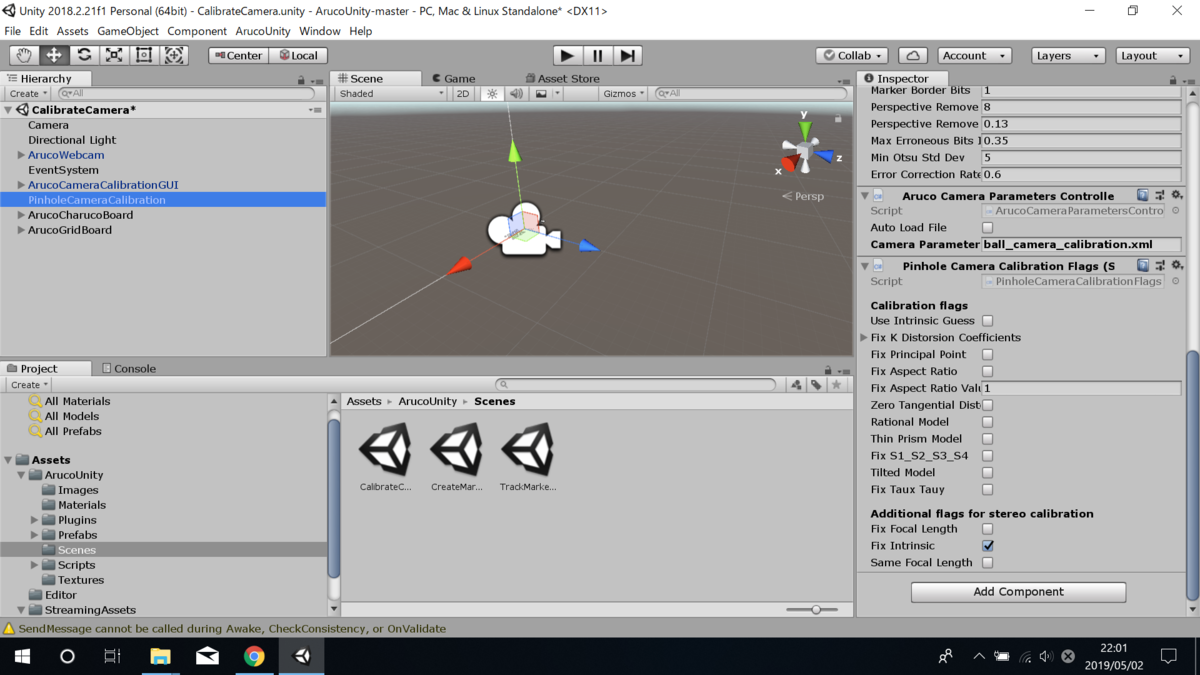カメラにボードを映して「add Image」を押すとキャリブレーション用のデータを取ることができます

これを10回程度繰り返して「Calibrate」を押すとキャリブレーションが実行されます

「Reset」ボタンを押すと最初から始めることができます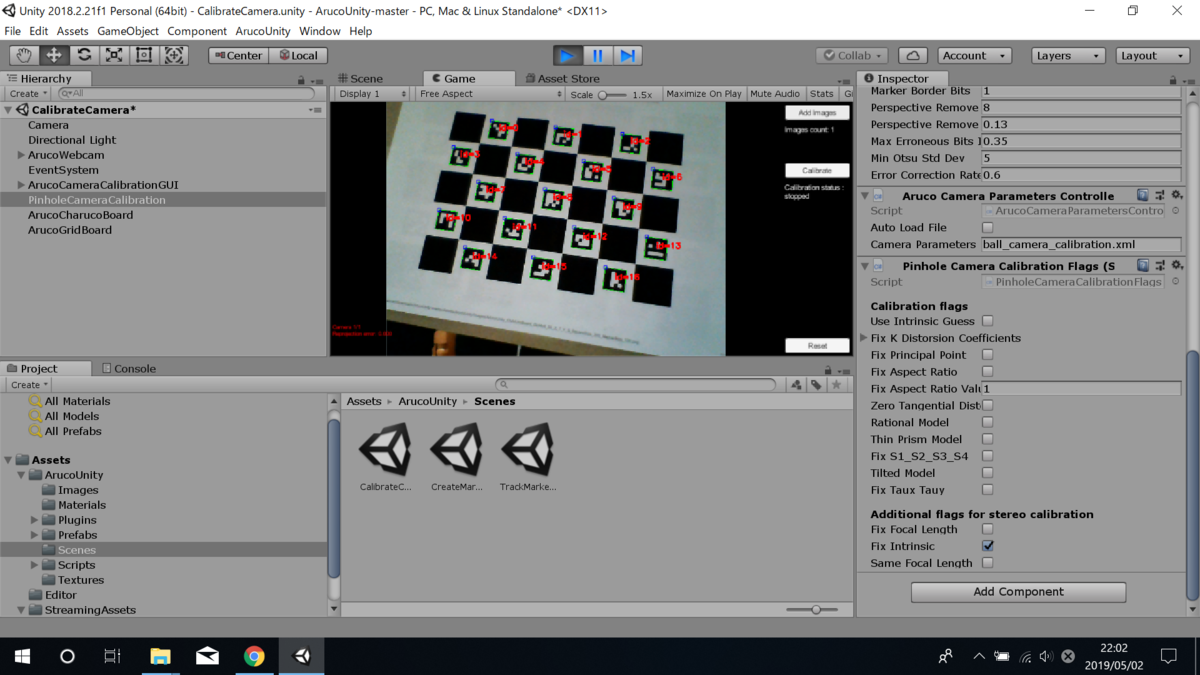(OpenCVではQRコードなしのキャリブレーション用ボードを用意しますが，今回の方法はQRコードがついています．これによってキャリブレーションボード全体が写っていなくてもキャリブレーションを行うことが出来るようです．ただ，QRコード全体が写っているほうが精度はもちろんいいです)

Assets/StreamingAssetsの中のxmlデータを確認します
estimatePoseSingleMarkersで必要なものは，Camera Matrices Value=cameraMatrix，DistCoeffsValues=distCoeffsです

cameraMatrix=
｜578・・，　0　 ，319・・｜
｜ 0　 ，581．．，259・・｜
｜ 0 ， 0 ， 1 ｜

distCoeffs=[0.0772，-0.2668，-0.0023，0.0024，-0.1549]

だと思います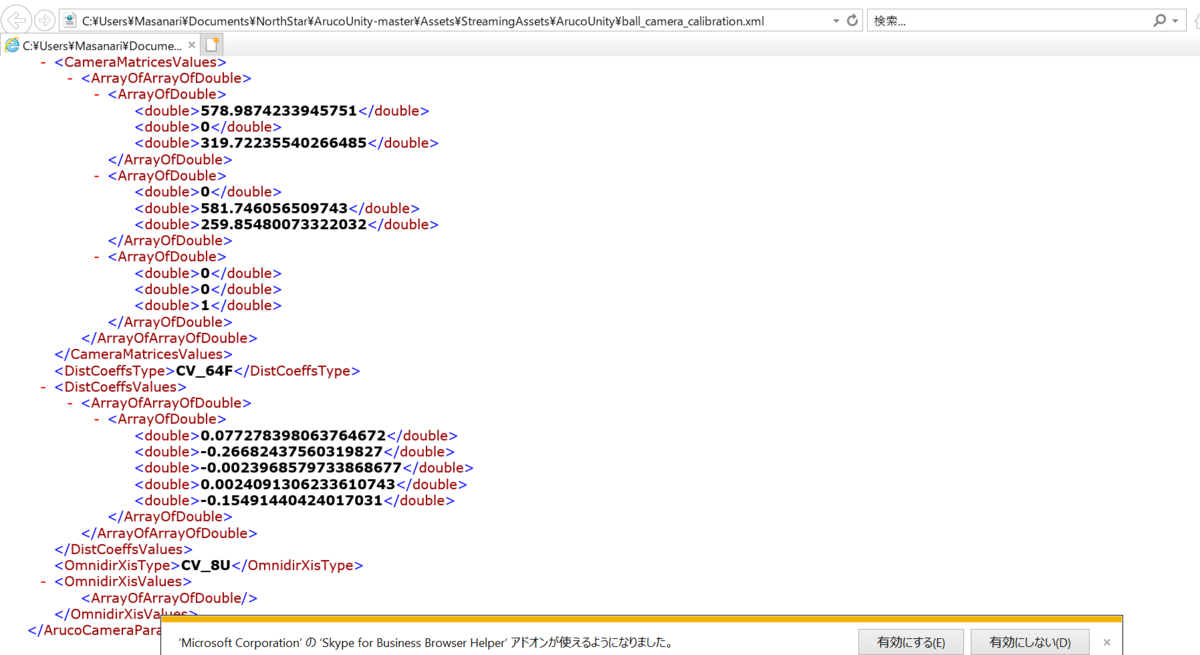これでカメラのキャリブレーションデータを取得することが出来ました

# 2019/5/3(UnityでArucoUnityを実行できる環境を作る)

mozyanari.hatenablog.com

ArUcoのライブラリの中に，estimatePoseSingleMarkersという関数があります
マーカーの姿勢と位置を計測できる関数で，ロボットにマーカを置いてロボットまでの相対位置を取得しようと考えています
しかし，この関数を実行するには，①InputArray cameraMatrix　②InputArray distCoeffsが必要です
docs.opencv.org

①は3×3のカメラ行列で，②は今回は1×5行列のレンズ歪みパラメータです
②はリファレンスによると1×4や1×12のものもあるらしいです

つまり，事前にこれらのパラメータを取得しないといけません

ですので，Unity上でこれらのパラメータを取得します

これらのプログラムを一から書くのはとても面倒なので今回は以下のプログラムを使いました
normanderwan.github.io

このプログラムはかなり優秀で，キャリブレーション用のボードを自作できたり，魚眼カメラ，ステレオカメラのキャリブレーションもできます
ただ，今回は普通のwebカメラキャリブレーションを最短で行うことを目標にします

• Unity 2018.2.21f1 (64-bit)
• ArucoUnity(Commits on Jan 12, 2019のものを使いました)
• ArucoUnityPlugin(ArucoUnityPlugin-v2.0.1-Windows.zipを使いました)

UnityでArucoUnityを実行できる環境を作る

github.com

UnityでArucoUnityを開きます
ただ，昔のバージョンで作られたので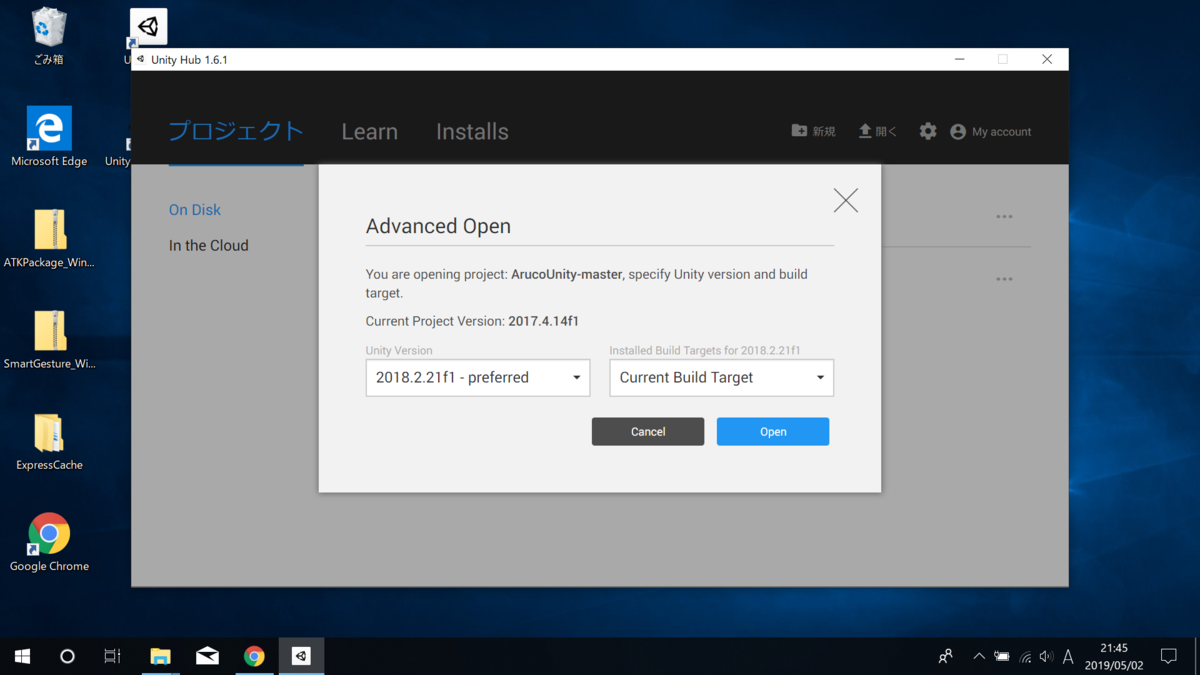このような警告が出ますが，2018のバージョンで開いても大丈夫なのでこのままオープンします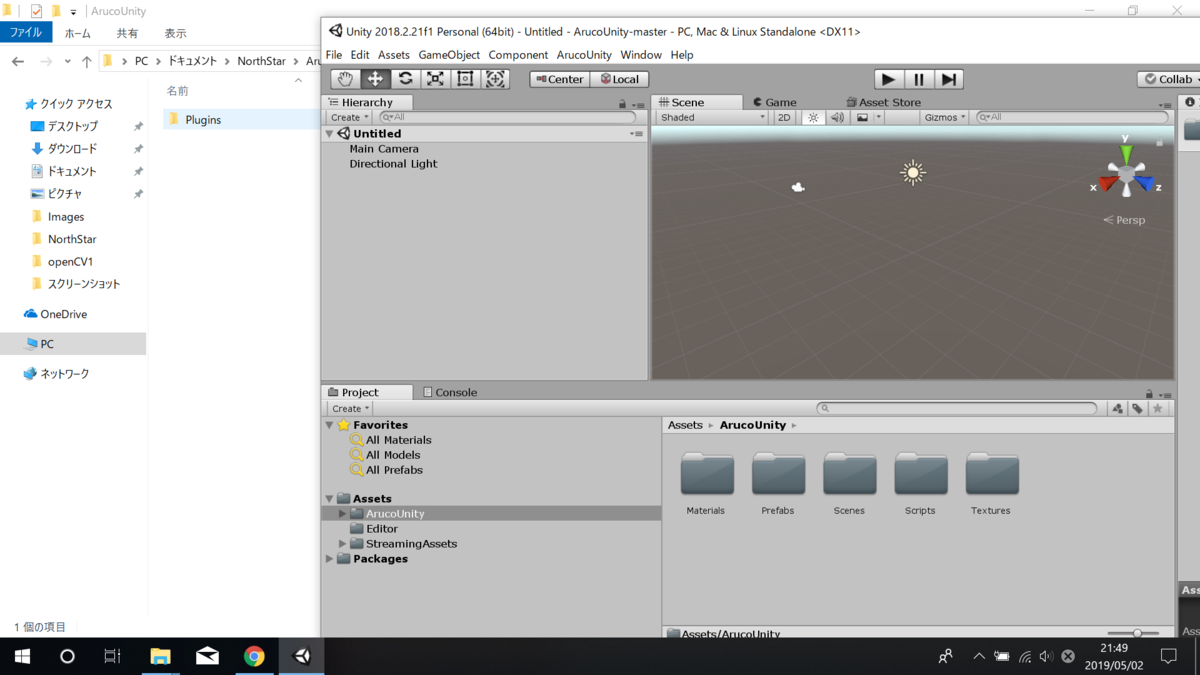ArucoUnityPluginの中のPluginsのフォルダを，UnityのAssets/ArucoUnityの中にドラッグ＆ドロップで入れます

すると，警告がすべてなくなっていると思います

# 2019/5/2(OpenCVでWebカメラの画像をモノクロにする)

GW中に高校の友達とご飯を食べに行ったときに，意外な進路をたどっていたので驚いた

mozyanari.hatenablog.com

```using System.Collections;
using System.Collections.Generic;
using UnityEngine;
using OpenCvSharp;

public class web_camera : MonoBehaviour {
[SerializeField] private int m_WebCamIndex = 1;
private Texture2D texture2D;
private VideoCapture videoCapture;
private Mat mat;
private Mat grey;

// Use this for initialization
void Start () {
videoCapture = new VideoCapture(m_WebCamIndex);
texture2D = new Texture2D(videoCapture.FrameWidth, videoCapture.FrameHeight, TextureFormat.RGB24, false);
GetComponent<Renderer>().material.mainTexture = texture2D;
mat = new Mat();
grey = new Mat();
}

// Update is called once per frame
void Update () {
videoCapture.Read(mat);
Cv2.CvtColor(mat, grey, ColorConversionCodes.BGR2GRAY);
texture2D.LoadImage(grey.ImEncode());
}

private void OnDestroy()
{
videoCapture.Dispose();
videoCapture = null;
}
}
```

startの部分

```videoCapture = new VideoCapture(m_WebCamIndex);
```

どのWebカメラを起動するかをここで選択して，カメラに関する情報を変数videoCaptureで取得します

```texture2D = new Texture2D(videoCapture.FrameWidth, videoCapture.FrameHeight, TextureFormat.RGB24, false);
```

カメラ画像に関する情報をtexture2Dで保持します
1つ目の引数で画像の横幅，2つ目で画像の縦幅を入力します
ここの引数をカメラの性能が分かっているなら決め打ちでもいいかもしれませんが，基本的にvideoCaptureから取得したほうが便利です
3つ目と4つ目はいまいち理解していません

```GetComponent<Renderer>().material.mainTexture = texture2D;
```

これを実行することでUnity上でアタッチしているオブジェクトにtexture2Dを張り付けることを示すことができます

```mat = new Mat();
grey = new Mat();
```

upadateの部分

```videoCapture.Read(mat);
```

videoCaptureにはwebカメラのデータが常に更新されて保存されているので，mat形式で書きだします

```Cv2.CvtColor(mat, grey, ColorConversionCodes.BGR2GRAY);
```

この部分がOpenCVの機能を使った部分で，変数matに入っているカメラ画像をモノクロ化(グレースケール化)して変数greyに格納しています

```texture2D.LoadImage(grey.ImEncode());
```

texture2Dはstartの部分でUnityのオブジェクトにアタッチされていることを示されているので，texture2Dに変数greyを入力するだけで勝手に更新されてUnity上で表示してくれます
ただ，greyはmat形式なのでImEncodeを使ってpngに変換してから入力します

OnDestroyの部分
この部分は参考にしたページには書いていましたが，自分の場合Unity上で実行した後この部分でエラーが発生したのでなくてもいいのかもしれません

これをUnityのPlaneにアタッチすれば，Plane上にwebカメラの画像が出てくるはずです

# 2019/4/29(UnityのOpenCVが動くかどうかを調べる)

前回の記事です

UnityでOpenCVが動く環境ができたのでとりあえず何か動かして，作った環境が正解か試します

1. 名前がTestのスクリプト作ります
2. 参考にしたページのプログラムをコピペして貼り付けます
3. てきとうなオブジェクトにアタッチして，実行すればプロジェクト内のフォルダに画像を生成する

しかし，ここで問題になることがありました

`var haarcascade = new CascadeClassifier("Assets/haarcascades/haarcascade_frontalface_default.xml");`

ここで，分類器？を参照しているのですが，自分が入れた環境ではそんなもの存在しておらず自分で入れる必要がありました

OpenCVの公式のsourceファイルにありました

ここでは，自分のOpenCVSharpのバージョンが4.0.0なので同じバージョンのソースをダウンロードしました

opencv-4.0.0\opencv-4.0.0\data\haarcascades\haarcascade_frontalface_default.xml

にありました

これをUnityの好きな場所にドラッグ＆ドロップで導入します

`var haarcascade = new CascadeClassifier("Assets/Scripts/haarcascade_frontalface_default.xml");`

に変更しました

この状態で実行すると，カメラが起動して結果の写真がプロジェクト内フォルダに出てきました

とりあえずこれで自分が作った環境がちゃんと動くものということが分かったのでこれで今後勉強していきます

# 2019/4/28(UnityにOpenCVSharp3を導入)

GW中はタケノコ掘り

UnityでOpenCVを使いたくて有志の方が制作したopencvsharp3を導入しました

ネットを参考にしながらやってみましたが、エラーが発生したのでそれに関して書いておきます

Unityのバージョンは，Unity 2018.2.21f1 (64-bit)です

• UnityにNuGetForUnity.1.1.0をインポート

• OpenCVSharp3をunityへインポート

そのあと，以下のリンクに従ってOpenCVSharp3を追加します

インポートすると以下のようなAssets/Packagesが入ります

(NuGetはNuGetForUnity.1.1.0.unitypackageをインポートしたときのものです)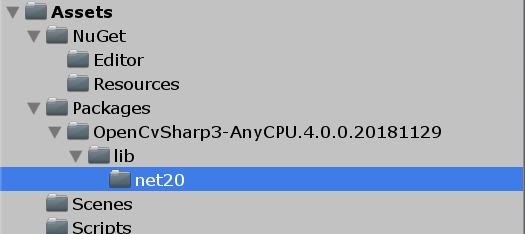そうすると，Matなどに代表されるOpenCVの標準的なライブラリが使えるようになると思います

さっきの参考にしたページをみて試しに適当なスクリプトを作るとMatが使えるようになっているはずです

• ArUcoなどの機能追加

なので，以下のブログを参考にしてスクリプトを書いてみました

けど，使おうとしたら以下のようなエラーが出ました

DllNotFoundException: OpenCvSharpExtern
OpenCvSharp.NativeMethods.LoadLibraries (IEnumerable`1 additionalPaths)
OpenCvSharp.NativeMethods..cctor ()
Rethrow as TypeInitializationException: An exception was thrown by the type initializer for OpenCvSharp.NativeMethods
OpenCvSharp.VideoCapture..ctor (Int32 index)
Rethrow as OpenCvSharpException: Failed to create VideoCapture
OpenCvSharp.VideoCapture..ctor (Int32 index)
webcam.Start () (at Assets/Scripts/webcam.cs:24)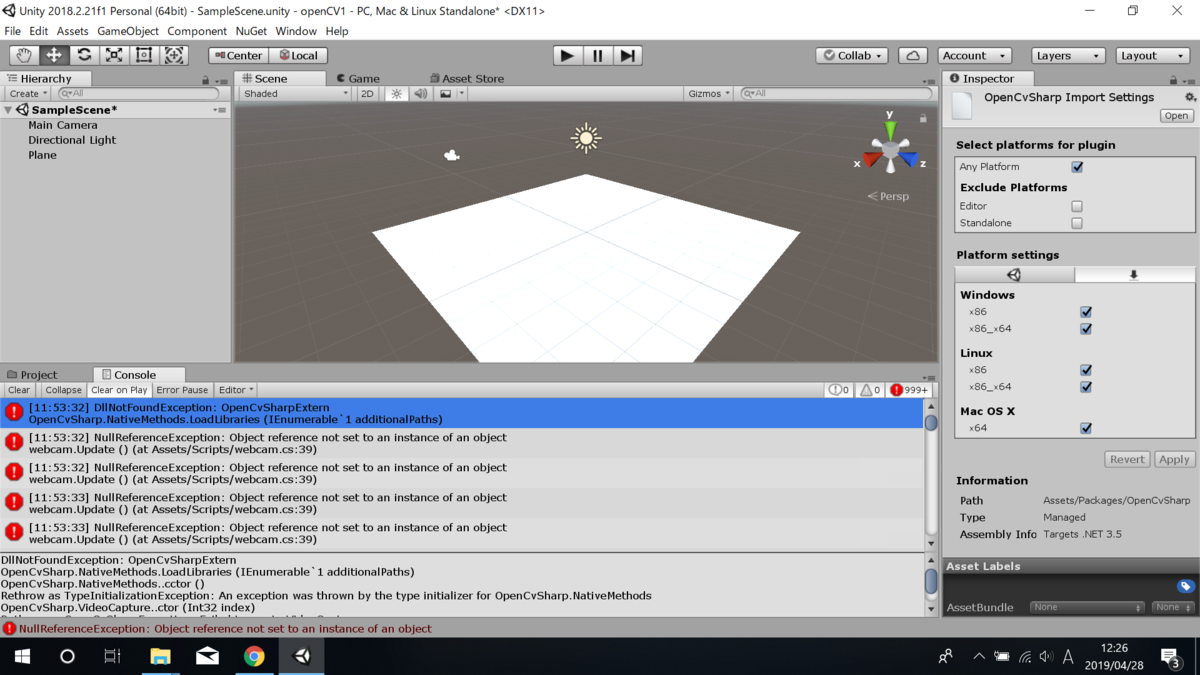OpenCvSharpExtern.dllが無いようです

というわけで，もしNuGetForUnityを使わずにOpenCVSharp3を入れるとどういうファイル構成になるかを調べるために以下のページからバージョン4.0.0のOpenCVSharp3をダウンロードしました

この時，x64の64ビットとx86の32ビットがありますが，Unityのバージョンに合わせてダウンロードしてください

つまり，UnityのバージョンとOpenCVのバージョンを合わせてダウンロードする必要があります

(もし，バージョン違いを入れるとエラー出ます)

そして，Unity経由で入るファイルと，製作者の人が出してるソースファイルを見るとOpenCvSharpExtern.dllが足りませんでした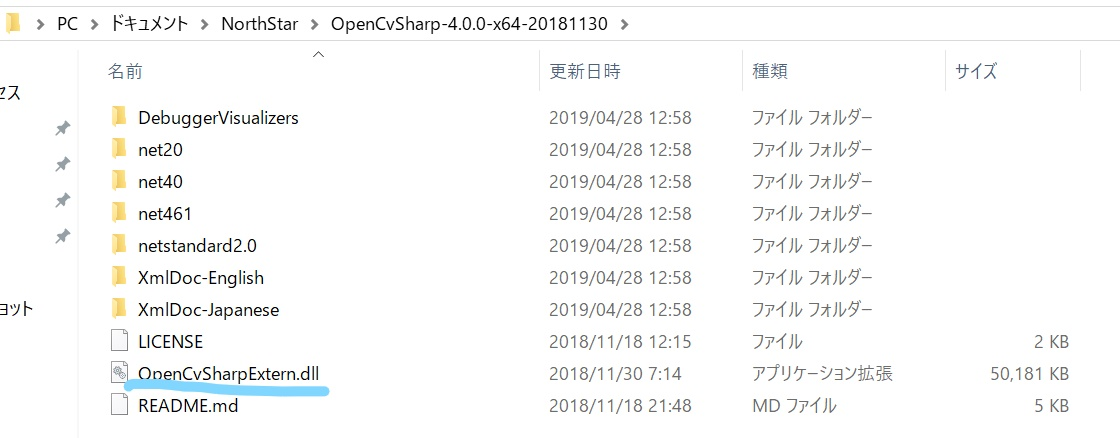なので，これをドラッグ＆ドロップでAssets/Packages/OpenCV..../libにOpenCvSharpExtern.dllをドラッグ＆ドロップで入れます

そうするとエラーがなくなりました

これで公式のOpenCVインストーラを使うことなくOpenCVをUnityに導入することができたはずです

# 2019/4/23(North Star製作日記 3.NorthStarが動く環境づくり)

ポルカのライブ良かった

ハードウェアが完成したので次は開発環境を整えます

まずはLeapmotionのSDKをダウンロードします

https://developer.leapmotion.com/get-started

ソフトウェア バージョン：4.0.0+52173

Firmware Revision: 1.7.0

です

NorthStarを動かすのに必要なソフトウェアは

この二つが必要です

ただ，何度かやってみた感じProjectNorthStarは最新版だと，LeapMotionのソフトウェアがまだ世に出てない最新バージョンを要求されて接続できませんでした

そのため

Unityのバージョンは現時点で最新であった，2019.1.0f2

ProjectNorthStarのバージョンは，Commits on Feb 21, 2019時点の物を使用しました

https://github.com/leapmotion/ProjectNorthStar/tree/a5002df12803a9165f73888080ada860342069f7

ここまで作れば参考にしたブログから動くようになると思います

これでNorthStarが動くようになりました

おおよそ一か月程度で製作することが出来ました